The Australian Journal of Mathematical Analysis and Applications

 Home News Editors Volumes RGMIA Subscriptions Authors Contact

ISSN 1449-5910

Sorry death is imminent for file: ../public_html/searchroot/files/tex/v19n2/v19i2p4.tex
You searched for rong
Total of 879 results found in site

42: Paper Source PDF document

Paper's Title:

Strong Convergence Theorems for a Common Zero of an Infinite Family of Gamma-Inverse Strongly Monotone Maps with Applications

Author(s):

Charles Ejike Chidume, Ogonnaya Michael Romanus, and Ukamaka Victoria Nnyaba

African University of Science and Technology, Abuja,
Nigeria.
E-mail: cchidume@aust.edu.ng
E-mail: romanusogonnaya@gmail.com
E-mail: nnyabavictoriau@gmail.com

Abstract:

Let E be a uniformly convex and uniformly smooth real Banach space with dual space E* and let Ak:EE*, k=1, 2, 3 , ...
be a family of inverse strongly monotone maps such that k=1 Ak-1(0)≠∅.
A new iterative algorithm is constructed and proved to converge strongly to a common zero of the family.
As a consequence of this result, a strong convergence theorem for approximating a common J-fixed point for an infinite family of
gamma-strictly J-pseudocontractive maps is proved. These results are new and improve recent results obtained for these classes of nonlinear maps.
Furthermore, the technique of proof is of independent interest.

33: Paper Source PDF document

Paper's Title:

Iterative Approximation of Zeros of Accretive Type Maps, with Applications

Author(s):

Charles Ejike Chidume, Chinedu Godwin Ezea, and Emmanuel Ezzaka Otubo

African University of Science and Technology, Abuja,
Nigeria.
E-mail: cchidume@aust.edu.ng
E-mail: chinedu.ezea@gmail.com
E-mail: mrzzaka@yahoo.com

Department of Mathematics,
Nnamdi Azikiwe University,
Awka,
Nigeria
E-mail: chinedu.ezea@gmail.com

Ebonyi State University,
Abakaliki,
Nigeria
E-mail: mrzzaka@yahoo.com

Abstract:

Let E be a reflexive real Banach space with uniformly Gâteaux differentiable norm. Let J:E E* be the normalized duality map on E and let A:E* E be a map such that AJ is an accretive and uniformly continuous map. Suppose that (AJ)-1(0) in nonempty. Then, an iterative sequence is constructed and proved to converge strongly to some u* in (AJ)-1(0). Application of our theorem in the case that E is a real Hilbert space yields a sequence which converges strongly to a zero of A. Finally, non-trivial examples of maps A for which AJ is accretive are presented..

31: Paper Source PDF document

Paper's Title:

Author(s):

F. U. Ogbuisi

School of Mathematics, Statistics and Computer Science,
University of KwaZulu-Natal, Durban,
South Africa.

Department of Mathematics,
University of Nigeria, Nsukka,
Nigeria.
E-mail: ferdinard.ogbuisi@unn.edu.ng fudochukwu@yahoo.com

Abstract:

In this paper, we propose and analyze a type of subgradient extragradient algorithm for the approximation of a solution of variational inequality problem which is also a common fixed point of an infinite family of relatively nonexpansive mappings in 2-uniformly convex Banach spaces which are uniformly smooth. By using the generalized projection operator, we prove a strong convergence theorem which does not require the prior knowledge of the Lipschitz constant of cost operator. We further applied our result to constrained convex minimization problem, convex feasibility problem and infinite family of equilibrium problems. Our results improve and complement related results in 2-uniformly convex and uniformly smooth Banach spaces and Hilbert spaces.

29: Paper Source PDF document

Paper's Title:

Strong Convergence Theorem for a Common Fixed Point of an Infinite Family of J-nonexpansive Maps with Applications

Author(s):

Charlse Ejike Chidume, Otubo Emmanuel Ezzaka and Chinedu Godwin Ezea

African University of Science and Technology,
Abuja,
Nigeria.
E-mail: cchidume@aust.edu.ng

Ebonyi State University,
Abakaliki,
Nigeria.
E-mail: mrzzaka@yahoo.com

Nnamdi Azikiwe University,
Awka,
Nigeria.
E-mail: chinedu.ezea@gmail.com

Abstract:

Let E be a uniformly convex and uniformly smooth real Banach space with dual space E*. Let {Ti}i=1 be a family of J-nonexpansive maps, where, for each i,~Ti maps E to 2E*. A new class of maps, J-nonexpansive maps from E to E*, an analogue of nonexpansive self maps of E, is introduced. Assuming that the set of common J-fixed points of {Ti}i=1 is nonempty, an iterative scheme is constructed and proved to converge strongly to a point x* in n=1FJTi. This result is then applied, in the case that E is a real Hilbert space to obtain a strong convergence theorem for approximation of a common fixed point for an infinite family of nonexpansive maps, assuming existences. The theorem obtained is compared with some important results in the literature. Finally, the technique of proof is also of independent interest.

29: Paper Source PDF document

Paper's Title:

MSplit Equality for Monotone Inclusion Problem and Fixed Point Problem in Real Banach Spaces

Author(s):

1,2Christian Chibueze Okeke, 3Abdumalik Usman Bello, 1Chinedu Izuchukwu, and 1Oluwatosin Temitope Mewomo

1School of Mathematics,
Statistics and Computer Science,
University of KwaZulu-Natal, Durban,
South Africa.
E-mail: okekec@ukzn.ac.za
E-mail: izuchukwuc@ukzn.ac.za
E-mail: mewomoo@ukzn.ac.za

2DST-NRF Center of Excellence in Mathematical and Statistical Sciences (CoE-Mass)
Johannesburg,
South Africa.

3Federal University,
Dutsin-Ma, Katsina State,
Nigeria.
E-mail: uabdulmalik@fudutsinma.edu.ng

Abstract:

In this paper a new iterative algorithm for approximating a common solution of split equality monotone inclusion problem and split equality fixed point problem is introduced. Using our algorithm, we state and prove a strong convergence theorem for approximating an element in the intersection of the set of solutions of a split equality monotone inclusion problem and the set of solutions of a split equality fixed point problem for right Bregman strongly nonexpansive mappings in the setting of p-uniformly convex Banach spaces which are also uniformly smooth. We also give some applications.

25: Paper Source PDF document

Paper's Title:

Iterative Algorithm for Split Generalized Mixed Equilibrium Problem Involving Relaxed Monotone Mappings in Real Hilbert Spaces

Author(s):

1U.A. Osisiogu, F.L. Adum, and 2C. Izuchukwu

1Department of Mathematics and Computer Science,
Ebonyi State University, Abakaliki,
Nigeria.

2School of Mathematics, Statistics and Computer Science,
University of KwaZulu-Natal, Durban,
South Africa.
E-mail: izuchukwuc@ukzn.ac.za, izuchukwu_c@yahoo.com

Abstract:

The main purpose of this paper is to introduce a certain class of split generalized mixed equilibrium problem involving relaxed monotone mappings. To solve our proposed problem, we introduce an iterative algorithm and obtain its strong convergence to a solution of the split generalized mixed equilibrium problems in Hilbert spaces. As special cases of the proposed problem, we studied the proximal split feasibility problem and variational inclusion problem.

24: Paper Source PDF document

Paper's Title:

On Convergence Theorems of an Implicit Iterative Process with Errors for a Finite Family of Asymptotically quasi I-nonexpansive Mappings

Author(s):

Farrukh Mukhamedov and Mansoor Saburov

Department of Computational & Theoretical Sciences,
Faculty of Sciences, International Islamic University Malaysia,
P.O. Box, 141, 25710, Kuantan,
Malaysia

farrukh_m@iiu.edu.my

msaburov@gmail.com

http://www.iium.edu.my

Abstract:

In this paper we prove the weak and strong convergence of the implicit iterative process with errors to a common fixed point of a finite family {Tj}Ni=1 of asymptotically quasi Ij-nonexpansive mappings as well as a family of {Ij}Nj=1 of asymptotically quasi nonexpansive mappings in the framework of Banach spaces. The obtained results improve and generalize the corresponding results in the existing literature.

23: Paper Source PDF document

Paper's Title:

Asymptotic Analysis of Positive Decreasing Solutions of a Class of Systems of Second Order Nonlinear Differential Equations in the Framework of Regular Variation

Author(s):

Jaroslav Jaroš, Kusano Takaŝi, Tomoyuki Tanigawa

Department of Mathematical Analysis and Numerical Mathematics,
Faculty of Mathematics, Physics and Informatics,
Comenius Universiy, 842 48 Bratislava,
Slovakia.

E-mail: ksntksjm4@gmail.com

Professor Emeritus at: Hiroshima University,
Department of Mathematics, Faculty of Science,
Higashi-Hiroshima 739-8526,
Japan.

E-mail: jaros@fmph.uniba.sk

Department of Mathematics, Faculty of Education,
Kumamoto University, Kumamoto 860-8555,
Japan.

E-mail: tanigawa@educ.kumamoto-u.ac.jp

Abstract:

The system of nonlinear differential equations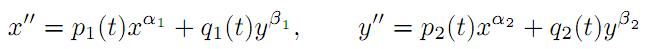is under consideration, where αi and βi are positive constants and pi(t) and qi(t) are continuous regularly varying functions on [a,). Two kinds of criteria are established for the existence of strongly decreasing regularly varying solutions with negative indices of (A) with precise asymptotic behavior at infinity. Fixed point techniques and basic theory of regular variation are utilized for this purpose.

23: Paper Source PDF document

Paper's Title:

A Self Adaptive Method for Solving Split Bilevel Variational Inequalities Problem in Hilbert Spaces

Author(s):

Francis Akutsah1, Ojen Kumar Narain2, Funmilayo Abibat Kasali3 Olawale Kazeem Oyewole4 and Akindele Adebayo Mebawondu5

1School of Mathematics,
Statistics and Computer Science,
University of KwaZulu-Natal, Durban,
South Africa.
E-mail: 216040405@stu.ukzn.ac.za, akutsah@gmail.com

2School of Mathematics,
Statistics and Computer Science,
University of KwaZulu-Natal, Durban,
South Africa.
E-mail: naraino@ukzn.ac.za

3Mountain Top University,
Prayer City, Ogun State,
Nigeria.
E-mail: fkasali@mtu.edu.ng

4Technion-Israel Institute of Technology.
E-mail: 217079141@stu.ukzn.ac.za, oyewoleolawalekazeem@gmail.co

5School of Mathematics,
Statistics and Computer Science,
University of KwaZulu-Natal, Durban,
South Africa.
DST-NRF Centre of Excellence in Mathematical and Statistical Sciences (CoE-MaSS),
Johannesburg,
South Africa.
Mountain Top University,
Prayer City, Ogun State,
Nigeria.
E-mail: dele@aims.ac.za

Abstract:

In this work, we study the split bilevel variational inequality problem in two real Hilbert spaces. We propose a new modified inertial projection and contraction method for solving the aforementioned problem when one of the operators is pseudomonotone and Lipschitz continuous while the other operator is α-strongly monotone. The use of the weakly sequential continuity condition on the Pseudomonotone operator is removed in this work. A Strong convergence theorem of the proposed method is proved under some mild conditions. In addition, some numerical experiments are presented to show the efficiency and implementation of our method in comparison with other methods in the literature in the framework of infinite dimensional Hilbert spaces. The results obtained in this paper extend, generalize and improve several.

21: Paper Source PDF document

Paper's Title:

On some Strongly Nonlinear Elliptic Problems in -data with a Nonlinearity Having a Constant Sign in Orlicz Spaces via Penalization Methods

Author(s):

E. Azroul, A. Benkirane and M. Rhoudaf

Dep. Math., Faculté des Sciences Dhar-Mahraz,
B.P 1796 Atlas Fčs,
Maroc

Departement of Mathematics,
Faculty of Sciences and Techniques of Tangier,
B.P. 416, Tangier,
Morocco.
rhoudaf_mohamed@yahoo.fr

Abstract:

This paper is concerned with the existence result of the unilateral problem associated to the equations of the type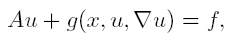in Orlicz spaces, without assuming the sign condition in the nonlinearity g. The source term f belongs to Lą(Ώ).

21: Paper Source PDF document

Paper's Title:

A New Method with Regularization for Solving Split Variational Inequality Problems in Real Hilbert Spaces

Author(s):

Francis Akutsah1 and Ojen Kumar Narain2

1School of Mathematics,
Statistics and Computer Science,
University of KwaZulu-Natal, Durban,
South Africa.
E-mail: 216040405@stu.ukzn.ac.za, akutsah@gmail.com

2School of Mathematics,
Statistics and Computer Science,
University of KwaZulu-Natal, Durban,
South Africa.
E-mail: naraino@ukzn.ac.za

Abstract:

In this paper, we introduce a new inertial extrapolation method with regularization for approximating solutions of split variational inequality problems in the frame work of real Hilbert spaces. We prove that the proposed method converges strongly to a minimum-norm solution of the problem without using the conventional two cases approach. In addition, we present some numerical experiments to show the efficiency and applicability of the proposed method. The results obtained in this paper extend, generalize and improve several results in this direction.

20: Paper Source PDF document

Paper's Title:

Strongly Nonlinear Variational Parabolic Problems in Weighted Sobolev Spaces

Author(s):

L. Aharouch, E. Azroul and M. Rhoudaf

Dép. Math. Faculté des Sciences Dhar-Mahraz
B.P 1796 Atlas Fés,
Maroc.
rhoudaf_mohamed@yahoo.fr

Abstract:

In this paper , we study the existence of a weak solutions for the initial-boundary value problems of the strongly nonlinear degenerated parabolic equation,

 ∂u +A(u)+g(x,t,u,∇ u)=f ∂t

where A is a Leray-lions operator acted from Lp(0,T,W01,p(Ώ,w)) into its dual. g(x,t,u,∇ u) is a nonlinear term with critical growth condition with respect to ∇ u and no growth with respect to u. The source term f is assumed to belong to Lp'(0,T,W-1,p'(Ώ,w*)).

19: Paper Source PDF document

Paper's Title:

Approximation of Common Fixed Points of a Finite Family of Asymptotically Demicontractive Mappings in Banach Spaces

Author(s):

Yuchao Tang, Yong Cai, Liqun Hu and Liwei Liu

Department of Mathematics, NanChang University,
Nanchang 330031, P.R. China
Department of Mathematics, Xi'an Jiaotong University,
Xi'an 710049, P.R. China

hhaaoo1331@yahoo.com.cn

Abstract:

By virtue of new analytic techniques, we analyze and study
several strong convergence theorems for the approximation of
common fixed points of asymptotically demicontractive mappings
via the multistep iterative sequence with errors in Banach
spaces. Our results improve and extend the corresponding ones
announced by Osilike , Osilike and Aniagbosor, Igbokwe, Cho et
al., Moore and Nnoli, Hu and all the others.

18: Paper Source PDF document

Paper's Title:

Existence of Bounded Solutions for a Class of Strongly Nonlinear Elliptic Equations in Orlicz-Sobolev Spaces

Author(s):

Abdelmoujib Benkirane and Ahmed Youssfi

Department of Mathematics and Informatics, Faculty of Sciences Dhar El Mahraz
University Sidi Mohammed Ben Abdallah
PB 1796 Fez-Atlas, Fez
Morocco
a.benkirane@menara.ma
ahmed.youssfi@caramail.com

Abstract:

We prove, in the setting of Orlicz-Sobolev spaces, the existence of bounded solutions for some strongly nonlinear elliptic equations with operator of the principal part having degenerate coercivity and lower order terms not satisfying the sign condition. The data have a suitable summability and no Δ2-condition is needed for the considered N-functions.

18: Paper Source PDF document

Paper's Title:

A Dynamic Contact Problem for an Electro Viscoelastic Body

Author(s):

Denche M. and Ait Kaki L.

Laboratoire Equations Differentielles,
Departement de Mathematiques,
Universite Constantine 1,
Algeria.

Ecole Normale Superieure,
Departement des Sciences Exactes et Informatique,
Plateau Mansourah, Constantine.
Algeria.

Abstract:

We consider a dynamic problem which describes a contact between a piezoelectric body and a conductive foundation. The frictionless contact is modelled with the normal compliance, the electric conditions are supposed almost perfect. We prove the existence of a unique weak solution for almost perfect electric contact.

18: Paper Source PDF document

Paper's Title:

Some Convergence Results for  Jungck-Am Iterative Process In Hyperbolic Spaces

Author(s):

Akindele Adebayo Mebawondu and Oluwatosin Temitope Mewomo

School of Mathematics, Statistics and Computer Science,
University of KwaZulu-Natal, Durban,
South Africa.
E-mail: 216028272@stu.ukzn.ac.za, mewomoo@ukzn.ac.za

Abstract:

In this paper, we introduce a new three steps iterative process called Jungck-AM iterative process and show that the proposed iterative process can be used to approximate fixed points of Jungck-contractive type mappings and Jungck-Suzuki type mappings. In addition, we establish some strong and Δ-convergence results for the approximation of fixed points of Jungck-Suzuki type mappings in the frame work of uniformly convex hyperbolic space. Furthermore, we show that the newly proposed iterative process has a better rate of convergence compare to the Jungck-Noor, Jungck-SP, Jungck-CR and some existing iterative processes in the literature. Finally, stability, data dependency results for Jungck-AM iterative process is established and we present an analytical proof and numerical examples to validate our claim.

18: Paper Source PDF document

Paper's Title:

Algorithms for Nonlinear Problems Involving Strictly Pseudocontractive Mappings

Author(s):

Mathew Olajiire Aibinu1, Surendra Colin Thakur2, Sibusiso Moyo3

1Institute for Systems Science & KZN E-Skill CoLab,
Durban University of Technology,
Durban 4000,
South Africa.

1DSI-NRF Centre of Excellence in Mathematical and Statistical Sciences (CoE-MaSS),
Johannesburg,
South Africa.
E-mail:
moaibinu@yahoo.com mathewa@dut.ac.za

2 KZN E-Skill CoLab,
Durban University of Technology,
Durban 4000,
South Africa.
E-mail:
thakur@dut.ac.za

3Institute for Systems Science & Office of the DVC Research, Innovation & Engagement Milena Court,
Durban University of Technology,
Durban 4000,
South Africa.
E-mail:
dvcrie@dut.ac.za

Abstract:

The puzzles in approximating a fixed point of nonlinear problems involving the class of strictly pseudocontractive mappings are conquered in this paper through viscosity implicit rules. Using generalized contraction mappings, a new viscosity iterative algorithm which is implicit in nature is proposed and analysed in Banach spaces for the class of strictly pseudocontractive mappings. The computations and analysis which are used in the proposed scheme are easy to follow and this gives rooms for a broad application of the scheme. It is obtained that the proposed iterative algorithm converges strongly to a fixed point of a μ-strictly pseudocontractive mapping which also solves a variational inequality problem. The result is also shown to hold for finite family of strictly pseudocontractive mappings. A numerical example is given to show the skillfulness of the proposed scheme and its implementation.

17: Paper Source PDF document

Paper's Title:

Applications of Relations and Relators in the Extensions of Stability Theorems for Homogeneous and Additive Functions

Author(s):

Árpád Száz

Institute of Mathematics, University of Debrecen,
H-4010 Debrecen, Pf. 12,
Hungary
szaz@math.klte.hu

Abstract:

By working out an appropriate technique of relations and relators and extending the ideas of the direct methods of Z. Gajda and R. Ger, we prove some generalizations of the stability theorems of D. H. Hyers, T. Aoki, Th. M. Rassias and P. Găvruţă in terms of the existence and unicity of 2-homogeneous and additive approximate selections of generalized subadditive relations of semigroups to vector relator spaces. Thus, we obtain generalizations not only of the selection theorems of Z. Gajda and R. Ger, but also those of the present author.

17: Paper Source PDF document

Paper's Title:

Convergence Speed of Some Random Implicit-Kirk-type Iterations for Contractive-type Random Operators

Author(s):

H. Akewe, K.S. Eke

Department of Mathematics,
Covenant University,
Canaanland, KM 10, Idiroko Road, P. M. B. 1023, Ota, Ogun State,
Nigeria.
E-mail: hudson.akewe@covenantuniversity.edu.ng, kanayo.eke@covenantuniversity.edu.ng

Abstract:

The main aim of this paper is to introduce a stochastic version of multistep type iterative scheme called a modified random implicit-Kirk multistep iterative scheme and prove strong convergence and stability results for a class of generalized contractive-type random operators. The rate of convergence of the random iterative schemes are also examined through an example. The results show that our new random implicit kirk multistep scheme perform better than other implicit iterative schemes in terms of convergence and thus have good potentials for further applications in equilibrium problems in computer science, physics and economics.

16: Paper Source PDF document

Paper's Title:

Iterative Approximation of Common Fixed Points of a Finite Family of Asymptotically Hemi-contractive Type Mappings

Author(s):

Jui-Chi Huang

Center for General Education,
Northern Taiwan Institute of Science and Technology,
Peito, Taipei,
Taiwan, 11202, R.O.C.
juichi@ntist.edu.tw

Abstract:

In this paper, we prove that the sequence of the modified Ishikawa-Xu,
Ishikawa-Liu, Mann-Xu and Mann-Liu iterative types of a finite family of
asymptotically hemi-contractive type mappings converges strongly to a common
fixed point of the family in a real p-uniformly convex Banach space with
p>1. Our results improve and extend some recent results.

15: Paper Source PDF document

Paper's Title:

On Vector Variational Inequality Problem in Terms of Bifunctions

Author(s):

C. S. Lalitha and Monika Mehta

Department of Mathematics, Rajdhani College,
University of Delhi, Raja Garden,
Delhi 110015, India
cslalitha@rediffmail.com

Department of Mathematics, Satyawati College,
University Of Delhi, Ashok Vihar,
Phase-III, Delhi 110052, India
mridul_in@yahoo.com

Abstract:

In this paper, we consider a generalized vector variational inequality problem expressed in terms of a bifunction and establish existence theorems for this problem by using the concepts of cone convexity and cone strong quasiconvexity and employing the celebrated Fan's Lemma. We also give two types of gap functions for this problem.

15: Paper Source PDF document

Paper's Title:

Shape Diagrams for 2D Compact Sets - Part II: Analytic Simply Connected Sets.

Author(s):

S. Rivollier, J. Debayle and J.-C. Pinoli

Ecole Nationale Supérieure des Mines de Saint-Etienne,

CIS - LPMG, UMR CNRS 5148, 158 cours Fauriel,

42023 Saint-Etienne Cedex 2, France.

Abstract:

Shape diagrams are representations in the Euclidean plane introduced to study 3-dimensional and 2-dimensional compact convex sets. However, they can also been applied to more general compact sets than compact convex sets. A compact set is represented by a point within a shape diagram whose coordinates are morphometrical functionals defined as normalized ratios of geometrical functionals. Classically, the geometrical functionals are the area, the perimeter, the radii of the inscribed and circumscribed circles, and the minimum and maximum Feret diameters. They allow twenty-two shape diagrams to be built. Starting from these six classical geometrical functionals, a detailed comparative study has been performed in order to analyze the representation relevance and discrimination power of these twenty-two shape diagrams. The first part of this study is published in a previous paper 16. It focused on analytic compact convex sets. A set will be called analytic if its boundary is piecewise defined by explicit functions in such a way that the six geometrical functionals can be straightforwardly calculated. The purpose of this paper is to present the second part, by focusing on analytic simply connected compact sets. The third part of the comparative study is published in a following paper 17. It is focused on convexity discrimination for analytic and discretized simply connected compact sets.

15: Paper Source PDF document

Paper's Title:

Shape Diagrams for 2D Compact Sets - Part III: Convexity Discrimination for Analytic and Discretized Simply Connected Sets.

Author(s):

S. Rivollier, J. Debayle and J.-C. Pinoli

Ecole Nationale Supérieure des Mines de Saint-Etienne,
CIS - LPMG, UMR CNRS 5148, 158 cours Fauriel,
42023 Saint-Etienne Cedex 2, France.

Abstract:

Shape diagrams are representations in the Euclidean plane introduced to study 3-dimensional and 2-dimensional compact convex sets. However, they can also been applied to more general compact sets than compact convex sets. A compact set is represented by a point within a shape diagram whose coordinates are morphometrical functionals defined as normalized ratios of geometrical functionals. Classically, the geometrical functionals are the area, the perimeter, the radii of the inscribed and circumscribed circles, and the minimum and maximum Feret diameters. They allow twenty-two shape diagrams to be built. Starting from these six classical geometrical functionals, a detailed comparative study has been performed in order to analyze the representation relevance and discrimination power of these twenty-two shape diagrams. The two first parts of this study are published in previous papers 8,9. They focus on analytic compact convex sets and analytic simply connected compact sets, respectively. The purpose of this paper is to present the third part, by focusing on the convexity discrimination for analytic and discretized simply connected compact sets..

15: Paper Source PDF document

Paper's Title:

Inclusion Properties of a Certain Subclass of Strongly Close-To-Convex Functions

Author(s):

S. M. Khairnar and M. More

Department of Mathematics,
Alandi -412 105, Pune, Maharashtra,
INDIA.

smkhairnar2007@gmail.com, meenamores@gmail.com.

Abstract:

The purpose of this paper is to derive some inclusion and argument properties of a new subclass of strongly close-to-convex functions in the open unit disc. We have considered an integral operator defined by convolution involving hypergeometric function in the subclass definition. The subclass also extends to the class of α-spirallike functions of complex order.

15: Paper Source PDF document

Paper's Title:

Generalizing Polyhedra to Infinite Dimension

Author(s):

Paolo d'Alessandro

Department of Mathematics, Third University of Rome,
Lgo S.L. Murialdo 1, 00146 Rome, Italy.

dalex@mat.uniroma3.it.

URL: http://www.mat.uniroma3.it/users/dalex/dalex.html.

Abstract:

This paper generalizes polyhedra to infinite dimensional Hilbert spaces as countable intersections of closed semispaces. Highlights are the structure theory that shows that a polyhedron is the sum of compact set (in a suitable topology) plus a closed pointed cone plus a closed subspace, giving the internal representation of polyhedra. In the final part the dual range space technique is extended to the solution of infinite dimensional LP problems.

15: Paper Source PDF document

Paper's Title:

Optimization and Approximation for Polyhedra in Separable Hilbert Spaces

Author(s):

Paolo d'Alessandro

Department of Mathematics,
Third University of Rome,
Italy.

E-mail: pdalex45@gmail.com

Abstract:

This paper studies infinite dimensional polyhedra, covering the case in which range spaces of operators defining inequality systems are not closed. A rangespace method of linear programming is generalized to infinite dimensions and finite dimensional methods of approximation are introduced.

14: Paper Source PDF document

Paper's Title:

New Implicit Kirk-Type Schemes for General Class of Quasi-Contractive Operators in Generalized Convex Metric Spaces

Author(s):

K. Rauf, O. T. Wahab and A. Ali

Department of Mathematics,
University of Ilorin, Ilorin,
Nigeria.
E-mail: krauf@unilorin.edu.ng

Department of Statistics and Mathematical Sciences,
Kwara State University, Malete,
Nigeria.

Department of Mathematics,
Mirpur University of Science and Technology, Mirpur,
Pakistan.

Abstract:

In this paper, we introduce some new implicit Kirk-type iterative schemes in generalized convex metric spaces in order to approximate fixed points for general class of quasi-contractive type operators. The strong convergence, T-stability, equivalency, data dependence and convergence rate of these results were explored. The iterative schemes are faster and better, in term of speed of convergence, than their corresponding results in the literature. These results also improve and generalize several existing iterative schemes in the literature and they provide analogues of the corresponding results of other spaces, namely: normed spaces, CAT(0) spaces and so on.

12: Paper Source PDF document

Paper's Title:

Polynomial Dichotomy of C0-Quasi Semigroups in Banach Spaces

Author(s):

Sutrima1,2, Christiana Rini Indrati2, Lina Aryati2

1Department of Mathematics,
Universitas Sebelas Maret,
PO Box 57126, Surakarta,
Indonesia.
E-mail: sutrima@mipa.uns.ac.id

2Department of Mathematics,
PO Box 55281, Yogyakarta,
Indonesia.
E-mail: rinii@ugm.ac.id, lina@ugm.ac.id

Abstract:

Stability of solutions of the problems is an important aspect for application purposes. Since its introduction by Datko , the concept of exponential stability has been developed in various types of stability by various approaches. The existing conditions use evolution operator, evolution semigroup, and quasi semigroup approach for the non-autonomous problems and a semigroup approach for the autonomous cases. However, the polynomial stability based on C0-quasi semigroups has not been discussed in the references. In this paper we propose a new stability for C0-quasi semigroups on Banach spaces i.e the polynomial stability and polynomial dichotomy. As the results, the sufficient and necessary conditions for the polynomial and uniform polynomial stability are established as well as the sufficiency for the polynomial dichotomy. The results are also confirmed by the examples.

12: Paper Source PDF document

Paper's Title:

Attempts to Define a Baum--Connes Map Via Localization of Categories for Inverse Semigroups

Author(s):

Bernhard Burgstaller

Departamento de Matematica,
CEP 88.040-900 Florianopolis-SC,
Brasil.
E-mail: bernhardburgstaller@yahoo.de
URL: http://mathematik.work/bernhardburgstaller/index.html

Abstract:

An induction functor in inverse semigroup equivariant KK-theory is considered, and together with %a restriction functors certain results similar to those known from the Mackey machinery are shown. It is also verified that for any so-called E-continuous inverse semigroup its equivariant KK-theory satisfies the universal property and is a triangulated category.

11: Paper Source PDF document

Paper's Title:

Shape Diagrams for 2D Compact Sets - Part I: Analytic Convex Sets.

Author(s):

S. Rivollier, J. Debayle and J.-C. Pinoli

Ecole Nationale Supérieure des Mines de Saint-Etienne,

CIS - LPMG, UMR CNRS 5148, 158 cours Fauriel,

42023 Saint-Etienne Cedex 2, France.

Abstract:

Shape diagrams are representations in the Euclidean plane introduced to study 3-dimensional and 2-dimensional compact convex sets. Such a set is represented by a point within a shape diagram whose coordinates are morphometrical functionals defined as normalized ratios of geometrical functionals. Classically, the geometrical functionals are the area, the perimeter, the radii of the inscribed and circumscribed circles, and the minimum and maximum Feret diameters. They allow thirty-one shape diagrams to be built. Most of these shape diagrams can also been applied to more general compact sets than compact convex sets. Starting from these six classical geometrical functionals, a detailed comparative study has been performed in order to analyze the representation relevance and discrimination power of these thirty-one shape diagrams. The purpose of this paper is to present the first part of this study, by focusing on analytic compact convex sets. A set will be called analytic if its boundary is piecewise defined by explicit functions in such a way that the six geometrical functionals can be straightforwardly calculated. The second and third part of the comparative study are published in two following papers [19.20]. They are focused on analytic simply connected sets and convexity discrimination for analytic and discretized simply connected sets, respectively.

11: Paper Source PDF document

Paper's Title:

Existence, Global Regularity and Uniqueness of Solutions of the Navier-Stokes Equations in Space Dimension 3 when the Initial Data are Regular

Author(s):

Moulay D. Tidriri

Email: mtctyasa@gmail.com

Abstract:

The existence, regularity, and uniqueness of global solutions of the Navier-Stokes equations in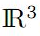are given for when the initial velocity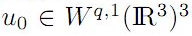for all integers q0 and div u0 = 0.

10: Paper Source PDF document

Paper's Title:

Fixed Point Theorems for a Finite Family of Asymptotically Nonexpansive Mappings

Author(s):

E. Prempeh

Department of Mathematics,
Kwame Nkrumah University of Science and Technology,
Kumasi, Ghana
edward_prempeh2000@yahoo.com

Abstract:

Let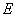be a real reflexive Banach space with a uniformly Gâteaux differentiable norm,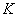be a nonempty bounded closed convex subset of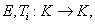i=1,2,...,r be a finite family of asymptotically nonexpansive mappings such that for each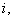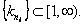Let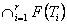be a nonempty set of common fixed points of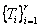and define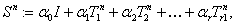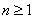. Let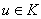be fixed and let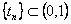be such that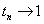as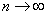. We can prove that the sequence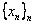satisfying the relation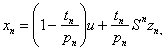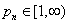associated with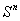, converges strongly to a fixed point of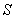providedpossesses uniform normal structure. Furthermore we prove that the iterative process: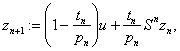, converges strongly to a fixed point of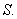10: Paper Source PDF document

Paper's Title:

Multivalued Hemiequilibrium Problems

Author(s):

Mathematics Department,
COMSATS Institute of Information Technology,
Pakistan.
noormaslam@hotmail.com

Abstract:

In this paper, we introduce and study a new class of equilibrium problems, known as multivalued hemiequilibrium problems. The auxiliary principle technique is used to suggest and analyze some new classes of iterative algorithms for solving multivalued hemiequilibrium problems. The convergence of the proposed methods either requires partially relaxed strongly monotonicity or pseudomonotonicity. As special cases, we obtain a number of known and new results for solving various classes of equilibrium and variational inequality problems. Since multivalued hemiequilibrium problems include hemiequilibrium, hemivariational inequalities, variational inequalities and complementarity problems as specials cases, our results still hold for these problems.

10: Paper Source PDF document

Paper's Title:

Merit Functions and Error Bounds for Mixed Quasivariational Inequalities

Author(s):

Mathematics Department, COMSATS Institute of Information Technology,
noormaslam@hotmail.com

Abstract:

It is well known that the mixed quasivariational inequalities are equivalent to the fixed point problems. We use this equivalent alternative formulation to construct some merit functions for mixed quasivariational inequalities and obtain error bounds under some conditions. Since mixed quasivariational inequalities include the classical variational inequalities and the complementarity problems as special cases, our results continue to hold for these problems.

10: Paper Source PDF document

Paper's Title:

Renormalized Solutions for Nonlinear Parabolic Equation with Lower Order Terms

Author(s):

A. Aberqi1, J. Bennouna1, M. Mekkour1 and H. Redwane2

1Université Sidi Mohammed Ben Abdellah,
Département de Mathématiques,
Laboratoire LAMA, Faculté des Sciences Dhar-Mahrez,
B.P 1796 Atlas Fés,
Morocco.

2Faculté des Sciences Juridiques, Economiques et Sociales,
Université Hassan 1, B.P. 784. Settat,
Morocco.

Abstract:

In this paper, we study the existence of renormalized solutions for the nonlinear parabolic problem: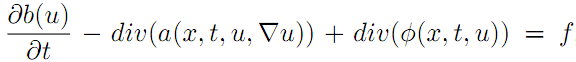,where the right side belongs to L1(Ω×(0,T)) and b(u) is unbounded function of u, the term div(a(x,t,u,u)) is a Leray--Lions operator and the function φ is a nonlinear lower order and satisfy only the growth condition.

9: Paper Source PDF document

Paper's Title:

Multivalued Equilibrium Problems with Trifunction

Author(s):

Etisalat College of Engineering, P.O. Box 980, Sharjah, United Arab Emirates
noor@ece.ac.ae

Abstract:

In this paper, we use the auxiliary principle technique to suggest some new classes of iterative algorithms for solving multivalued equilibrium problems with trifunction. The convergence of the proposed methods either requires partially relaxed strongly monotonicity or pseudomonotonicity. As special cases, we obtain a number of known and new results for solving various classes of equilibrium and variational inequality problems. Since multivalued equilibrium problems with trifunction include equilibrium, variational inequality and complementarity problems as specials cases, our results continue to hold for these problems.

9: Paper Source PDF document

Paper's Title:

Existence of solutions for Neutral Stochastic Functional Differential Systems with Infinite Delay in Abstract Space

Author(s):

P. Balasubramaniam, A. V. A. Kumar and S. K. Ntouyas

Department of Mathematics, Gandhigram Rural Institute,
Deemed University, Gandhigram - 624 302, Tamil Nadu, India.
pbalgri@rediffmail.com

Department of Mathematics, Gandhigram Rural Institute,
Deemed University, Gandhigram - 624 302, Tamil Nadu, India.
nnddww@tom.com

Department of Mathematics, University of Ioannina,
451 10 Ioannina,
Greece.
sntouyas@cc.uoi.gr
URL: http://www.math.uoi.gr/~sntouyas

Abstract:

In this paper we prove existence results for semilinear stochastic neutral functional differential systems with unbounded delay in abstract space. Our theory makes use of analytic semigroups and fractional power of closed operators and Sadovskii fixed point theorem.

7: Paper Source PDF document

Paper's Title:

Long Time Behavior for a Viscoelastic Problem with a Positive Definite Kernel

Author(s):

Nasser-eddine Tatar

King Fahd University of Petroleum and Mineral, Department of Mathematical Sciences,
Dhahran, 31261 Saudi Arabia

Abstract:

We study the asymptotic behavior of solutions for an integro-differential problem which arises in the theory of viscoelasticity. It is proved that solutions go to rest in an exponential manner under new assumptions on the relaxation function in the memory term. In particular, we consider a new family of kernels which are not necessarily decreasing.

7: Paper Source PDF document

Paper's Title:

On a Criteria for Strong Starlikeness

Author(s):

V. Ravichandran, M. Darus, and N. Seenivasagan

School Of Mathematical Sciences, Universiti Sains Malaysia,
11800 Usm Penang, Malaysia
vravi@cs.usm.my
URL: http://cs.usm.my/~vravi

School of Mathematical Sciences, Faculty of Sciences and Technology,
Ukm, Bangi 43600, Malaysia
maslina@pkrisc.cc.ukm.my

Sindhi College, 123, P. H. Road, Numbal,
Chennai 600 077 India
vasagan2000@yahoo.co.in

Abstract:

In this paper, we are concerned with finding sufficient condition for certain normalized analytic function f(z) defined on the open unit disk in the complex plane to be strongly starlike of order α. Also we have obtained similar results for certain functions defined by Ruscheweyh derivatives and Sălăgean derivatives. Further extension of these results are given for certain p-valent analytic functions defined through a linear operator.

6: Paper Source PDF document

Paper's Title:

Topological Aspects of Scalarization in Vector Optimization Problems.

Author(s):

Peter I. Kogut, Rosanna Manzo and Igor V. Nechay

Department of Differential Equations,
Dnipropetrovsk National University, Naukova STR.,
13, 49010 Dnipropetrovsk,
Ukraine

p.kogut@i.ua

Universitŕ di Salerno,
Dipartimento di Ingegneria dell'Informazione e Matematica Applicata,
Via Ponte don Melillo, 84084 Fisciano (SA),
Italy

manzo@diima.unisa.it

Department of Technical Cybernetics,
Dnipropetrovsk Technical University,
Acad. Lazarjan STR., 2, 49010 Dnipropetrovsk,
Ukraine

i.nechay@i.ua

Abstract:

In this paper, we study vector optimization problems in partially ordered Banach spaces. We suppose that the objective mapping possesses a weakened property of lower semicontinuity and make no assumptions on the interior of the ordering cone. We derive sufficient conditions for existence of efficient solutions of the above problems and discuss the role of topological properties of the objective space. We discuss the scalarization of vector optimization problems when the objective functions are vector-valued mappings with a weakened property of lower semicontinuity. We also prove the existence of the so-called generalized efficient solutions via the scalarization process. All principal notions and assertions are illustrated by numerous examples.

6: Paper Source PDF document

Paper's Title:

Inequalities for Functions of Selfadjoint Operators on Hilbert Spaces:
a Survey of Recent Results

Author(s):

Sever S. Dragomir1,2

1Mathematics, College of Engineering & Science
Victoria University, PO Box 14428
Melbourne City, MC 8001,
Australia
E-mail: sever.dragomir@vu.edu.au

2DST-NRF Centre of Excellence in the Mathematical and Statistical Sciences,
School of Computer Science & Applied Mathematics,
University of the Witwatersrand,
Private Bag 3, Johannesburg 2050,
South Africa
URL: https://rgmia.org/dragomir

Abstract:

The main aim of this survey is to present recent results concerning inequalities for continuous functions of selfadjoint operators on complex Hilbert spaces. It is intended for use by both researchers in various fields of Linear Operator Theory and Mathematical Inequalities, domains which have grown exponentially in the last decade, as well as by postgraduate students and scientists applying inequalities in their specific areas.

6: Paper Source PDF document

Paper's Title:

D-Iterative Method for Solving a Delay Differential Equation and a Two-Point Second-Order Boundary Value Problems in Banach Spaces

Author(s):

Francis Akutsah1, Akindele Adebayo Mebawondu2, Oluwatosin Babasola3, Paranjothi Pillay4 and Ojen Kumar Narain5

1School of Mathematics,
Statistics and Computer Science,
University of KwaZulu-Natal, Durban,
South Africa.
E-mail: 216040405@stu.ukzn.ac.za, akutsah@gmail.com

2School of Mathematics,
Statistics and Computer Science,
University of KwaZulu-Natal, Durban,
South Africa.
DST-NRF Centre of Excellence in Mathematical and Statistical Sciences (CoE-MaSS),
Johannesburg,
South Africa.
Mountain Top University,
Prayer City, Ogun State,
Nigeria.
E-mail: dele@aims.ac.za

3Department of Mathematical Sciences,
University of Bath,
Claverton Down,
Bath, BA2 7AY
UK.
E-mail: ob377@bath.ac.uk

4School of Mathematics,
Statistics and Computer Science,
University of KwaZulu-Natal, Durban,
South Africa.
E-mail: pillaypi@ukzn.ac.za

5School of Mathematics,
Statistics and Computer Science,
University of KwaZulu-Natal, Durban,
South Africa.
E-mail: naraino@ukzn.ac.za

Abstract:

The purpose of this paper is to re-establish the convergence, stability and data dependence results established by  and  by removing the strong assumptions imposed on the sequences which were used to obtain their results. In addition, we introduced a modified approach using the D-iterative method to solve a two-point second-order boundary value problem, and also obtain the solution of a delay differential equations using the obtained results in this paper. The results presented in this paper do not only extend and improve the results obtained in [2, 3], it further extends and improve some existing results in the literature.

5: Paper Source PDF document

Paper's Title:

On Sufficient Conditions for Strong Starlikeness

Author(s):

V. Ravichandran, M. H. Khan, M. Darus, And K. G. Subramanian

School of Mathematical Sciences, Universiti Sains Malaysia, 11800 Usm Penang, Malaysia
Url
: h
ttp://cs.usm.my/~vravi/index.html

Department of Mathematics, Islamiah College, Vaniambadi 635 751, India
khanhussaff@yahoo.co.in

School of Mathematical Sciences, Faculty of Science and Technology, UKM, Bangi 43600,
M
alaysia
maslina@pkrisc.cc.ukm.my
Url
:

Department of Mathematics, Madras Christian College, Tambaram, Chennai 600 059, India

Abstract:

In the present investigation, we obtain some sufficient conditions for a normalized analytic function f(z) defined on the unit disk to satisfy the condition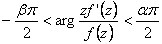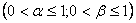5: Paper Source PDF document

Paper's Title:

Differentiability of Distance Functions in p-Normed Spaces

Author(s):

M. S. Moslehian, A. Niknam, S. Shadkam Torbati

Department of Pure Mathematics, Centre of Excellence in Analysis on Algebraic Structures (CEAAS),,
Iran

moslehian@ferdowsi.um.ac.ir
niknam@math.um.ac.ir

Abstract:

The farthest point mapping in a p-normed space X is studied in virtue of the Gateaux derivative and the Frechet derivative. Let M be a closed bounded subset of X having the uniformly p-Gateaux differentiable norm. Under certain conditions, it is shown that every maximizing sequence is convergent, moreover, if M is a uniquely remotal set then the farthest point mapping is continuous and so M is singleton. In addition, a Hahn--Banach type theorem in \$p\$-normed spaces is proved.

5: Paper Source PDF document

Paper's Title:

Generalized Efficient Solutions to One Class of Vector Optimization Problems in Banach Space

Author(s):

Peter I. Kogut, Rosanna Manzo, and Igor V. Nechay

Department of Differential Equations,
Dnipropetrovsk National University,
Naukova str., 13, 49050 Dnipropetrovsk,
Ukraine
p.kogut@i.ua

Dipartimento di Ingegneria Dell’informazione e Matematica Applicata,
Universitŕ di Salerno,
Via Ponte Don Melillo, 84084 Fisciano (Sa),
Italy
manzo@diima.unisa.it

Department of Technical Cybernetics,
Dnipropetrovsk Technical University,
Dnipro
petrovsk,
Ukraine
i.nechay@i.ua

Abstract:

In this paper, we study vector optimization problems in Banach spaces for essentially nonlinear operator equations with additional control and state constraints. We assume that an objective mapping possesses a weakened property of lower semicontinuity and make no assumptions on the interior of the ordering cone. Using the penalization approach we derive both sufficient and necessary conditions for the existence of efficient solutions of the above problems. We also prove the existence of the so-called generalized efficient solutions via the scalarization of some penalized vector optimization problem.

5: Paper Source PDF document

Paper's Title:

Generalized k-distance-balanced Graphs

Author(s):

Amir Hosseini and Mehdi Alaeiyan

Department of mathematics, Karaj Branch,
Iran.
E-mail: amir.hosseini@kiau.ac.ir, hosseini.sam.52@gmail.com

Department of Mathematics,
Iran University of Science and Technology, Tehran,
Iran.
E-mail: alaeiyan@iust.ac.ir

Abstract:

A nonempty graph Γ is called generalized k-distance-balanced, whenever every edge ab has the following property: the number of vertices closer to a than to b, k, times of vertices closer to b than to a, or conversely, k N .In this paper we determine some families of graphs that have this property, as well as to prove some other result regarding these graphs.

5: Paper Source PDF document

Paper's Title:

A Multivalued Version of the Radon-Nikodym Theorem, via the Single-valued Gould Integral

Author(s):

Domenico Candeloro1, Anca Croitoru2, Alina Gavriluţ2, Anna Rita Sambucini1

1Dept. of Mathematics and Computer Sciences,
University of Perugia,
1, Via Vanvitelli -- 06123, Perugia,
Italy.
E-mail:  domenico.candeloro@unipg.it, anna.sambucini@unipg.it

2Faculty of Mathematics,
Al. I. Cuza University,
700506 Iaşi,
Romania.
E-mail: croitoru@uaic.ro, gavrilut@uaic.ro

Abstract:

In this paper we consider a Gould type integral of real functions with respect to a compact and convex valued not necessarily additive measure. In particular we will introduce the concept of integrable multimeasure and, thanks to this notion, we will establish an exact Radon-Nikodym theorem relative to a fuzzy multisubmeasure which is new also in the finite dimensional case. Some results concerning the Gould integral are also obtained.

5: Paper Source PDF document

Paper's Title:

Some criteria for Subspace-hypercyclicity of C0-semigroups

Author(s):

Mansooreh Moosapoor

Department of Mathematics,
Farhangian University, Tehran,
Iran.
E-mail: m.mosapour@cfu.ac.ir
mosapor110@gmail.com

Abstract:

We research subspace-hypercyclic C0-semigroups in this paper. We present various types of subspace-hypercyclicity criteria for C0-semigroups. Some of them are stronger than the criteria introduced before. Also, we state that if a C0-semigroup (Tt}t 0 satisfies in any of them, then (Tt⊕Tt}t 0 is subspace-hypercyclic.

5: Paper Source PDF document

Paper's Title:

Nonlinear System of Mixed Ordered Variational Inclusions Involving XOR Operation

Author(s):

Iqbal Ahmad, Abdullah and Syed Shakaib Irfan

Department of Mechanical Engineering,
College of Engineering, Qassim University
Buraidah 51452, Al-Qassim,
Saudi Arabia.

Zakir Husain Delhi College,
University of Delhi,
JLN Marg, New Delhi- 110 002,
India.
E-mail: abdullahdu@qec.edu.sa

Department of Mathematics,
Aligarh Muslim University, Aligarh,
India.
E-mail: shakaibirfan@gmail.com

Abstract:

In this work, we introduce and solve an NSMOVI frameworks system involving XOR operation with the help of a proposed iterative algorithm in real ordered positive Hilbert spaces. We discuss the existence of a solution of a considered system of inclusions involving XOR operation by applying the resolvent operator technique with XOR operation and also study the strong convergence of the sequences generated by the considered algorithm. Further, we give a numerical example in support of our considered problem which gives the grantee that all the proposed conditions of our main result are fulfilled.

4: Paper Source PDF document

Paper's Title:

On Interaction of Discontinuous Waves in a Gas with Dust Particles

Author(s):

J. Jena

Department of Mathematics, Netaji Subhas institute of technology,
Sector-3, Dwarka, New Delhi - 110 075,
India.
jjena67@rediffmail.com
jjena@nsit.ac.in

Abstract:

In this paper, the interaction of the strong shock with the weak discontinuity has been investigated for the system of partial differential equations describing one dimensional unsteady plane flow of an inviscid gas with large number of dust particles. The amplitudes of the reflected and transmitted waves after interaction of the weak discontinuity through a strong shock are evaluated by exploiting the results of general theory of wave interaction.

4: Paper Source PDF document

Paper's Title:

The Convergence of Modified Mann-Ishikawa Iterations when Applied to an Asymptotically Pseudocontractive Map

Author(s):

S. Soltuz

Departamento de Matematicas, Universidad de Los Andes, Carrera 1
No. 18A-10, Bogota,
Colombia
and
``T. Popoviciu" Institute of Numerical Analysis
Cluj-Napoca,
Romania
smsoltuz@gmail.com
URL:http://www.uniandes.edu.co/

Abstract:

We prove that under minimal conditions the modified Mann and Ishikawa iterations converge when dealing with an asymptotically pseudocontractive map. We give an affirmative answer to the open question from C.E. Chidume and H. Zegeye, Approximate fixed point sequences and convergence theorems for asymptotically pseudocontractive mappings, J. Math. Anal. Appl., 278 (2003), 354--366.

4: Paper Source PDF document

Paper's Title:

Some Generalized Difference Sequence Spaces Defined by Orlicz Functions

Author(s):

Ramzi S. N. Alsaedi and Ahmad H. A. Bataineh

Department of Mathematics, King Abdul Aziz University,
Jeddah P.O.Box 80203,
Saudia Arabia
ramzialsaedi@yahoo.co.uk

Department of Mathematics, Al al-Bayt University,
Mafraq 25113,
Jordan
ahabf2003@yahoo.ca

Abstract:

In this paper, we define the sequence spaces: [V,M,p,u,Δ ],[V,M,p,u,Δ]0 and [V,M,p,u,Δ], where for any sequence x=(xn), the difference sequence Δx is given by Δx=(Δxn)= (xn-xn-1). We also study some properties and theorems of these spaces. These are generalizations of those defined and studied by Savas and Savas and some others before.

4: Paper Source PDF document

Paper's Title:

Maximal Inequalities for Multidimensionally Indexed Demimartingales and the Hájek-Rényi Inequality for Associated Random Variables

Author(s):

Tasos C. Christofides and Milto Hadjikyriakou

Department of Mathematics and Statistics
University of Cyprus
P.O.Box 20537, Nicosia 1678, Cyprus

tasos@ucy.ac.cy
miltwh@gmail.com

Abstract:

Demimartingales and demisubmartingales introduced by Newman and
Wright (1982) generalize the notion of martingales and
submartingales respectively. In this paper we define
multidimensionally indexed demimartingales and demisubmartingales
and prove a maximal inequality for this general class of random
variables. As a corollary we obtain a Hájek-Rényi inequality
for multidimensionally indexed associated random variables, the bound of which, when reduced to the case of single index, is sharper than the bounds already known in the literature.

4: Paper Source PDF document

Paper's Title:

New Solutions to Non-Smooth PDEs

Author(s):

Moawia Alghalith

University of the West Indies,
St. Augustine,

moawia.alghalith@sta.uwi.edu

Abstract:

We provide strong solutions to partial differential equations when the function is non-differentiable.

4: Paper Source PDF document

Paper's Title:

On the Regularization of Hammerstein's type Operator Equations

Author(s):

E. Prempeh, I. Owusu-Mensah and K. Piesie-Frimpong

Department of Mathematics,
KNUST, Kumasi-
Ghana.

Department of Science Education,
University of Education,
Winneba

Department of Mathematics,
Presbyterian University College, Abetifi,
Ghana

E-mail: isaacowusumensah@gmail.com

E-mail: eprempeh.cos@knust.edu.gh

E-mail: piesie74@yahoo.com

Abstract:

We have studied Regularization of Hammerstein's Type Operator Equations in general Banach Spaces. In this paper, the results have been employed to establish regularized solutions to Hammerstein's type operator equations in Hilbert spaces by looking at three cases of regularization.

4: Paper Source PDF document

Paper's Title:

On the Biharmonic Equation with Nonlinear Boundary Integral Conditions

Author(s):

R. Hamdouche and H. Saker

L.M.A. Department of Mathematics, Faculty of Sciences,
P.O.Box 12. Annaba 23000,
Algeria.
E-mail: h_saker@yahoo.fr, hmdch.rahma16@gmail.com

Abstract:

In the present work, we deal with the biharmonic problems in a bounded domain in the plane with the nonlinear boundary integral conditions. After applying the Boundary integral method, a system of nonlinear boundary integral equations is obtained. The result show that when the nonlinearity satisfies some conditions lead the existence and uniqueness of the solution.

3: Paper Source PDF document

Paper's Title:

Reverses of the CBS Integral Inequality in Hilbert Spaces and Related Results

Author(s):

I. Brnetić, S. S. Dragomir, R. Hoxha and J. Pečarić

Department of Applied Mathematics, Faculty of Electrical Engineering and Computing,
University of Zagreb, Unska 3, 10 000 Zagreb,
Croatia
andrea@zpm.fer.hr

School of Computer Science & Mathematics, Victoria University
Po Box 14428, Melbourne Vic 8001
Australia
sever.dragomir@vu.edu.au
URL:http://rgmia.vu.edu.au/dragomir

Faculty of Applied Technical Sciences, University of Prishtina,
Mother Theresa 5, 38 000 Prishtina
Kosova
razimhoxha@yahoo.com

Faculty of Textile Technology, University of Zagreb,
Pierottijeva 6, 10000 Zagreb,
Croatia
pecaric@hazu.hr

Abstract:

There are many known reverses of the Cauchy-Bunyakovsky-Schwarz (CBS) inequality in the literature. We obtain here a general integral inequality comprising some of those results and also provide other related inequalities. The discrete case, which is of interest in its own turn, is also analysed.

3: Paper Source PDF document

Paper's Title:

Some Properties of the Solution of a Second Order Elliptic Abstract Differential Equation

Author(s):

A. Aibeche and K. Laidoune

Mathematics Department, Faculty of Sciences, University Ferhat Abbas, Setif,
Route de Scipion, 19000, Setif,
Algeria
aibeche@univ-setif.dz

Abstract:

In this paper we study a class of non regular boundary value problems for elliptic differential-operator equation of second order with an operator in boundary conditions. We give conditions which guarantee the coerciveness of the solution of the considered problem, the completeness of system of root vectors in Banach-valued functions spaces and we establish the Abel basis property of this system in Hilbert spaces. Finally, we apply this abstract results to a partial differential equation in cylindrical domain.

3: Paper Source PDF document

Paper's Title:

Improvement of Jensen's Inequality for Superquadratic Functions

Author(s):

S. Abramovich, B. Ivanković, and J. Pečarić

Department of Mathematics,
University of Haifa,
Haifa 31905,
Israel.
abramos@math.haifa.ac.il

Faculty of Transport and Trafic Engineering,
University of Zagreb,
Vukelićeva 4, 10000,
Croatia
bozidar.ivankovic@zg.t-com.hr

Faculty of Textile,
University of Zagreb,
Prilaz Baruna Filipovića 30, 10000 Zagreb,
Croatia
pecaric@element.hr

Abstract:

Since 1907, the famous Jensen's inequality has been refined in different manners. In our paper, we refine it applying superquadratic functions and separations of domains for convex functions. There are convex functions which are not superquadratic and superquadratic functions which are not convex. For superquadratic functions which are not convex we get inequalities analogue to inequalities satisfied by convex functions. For superquadratic functions which are convex (including many useful functions) we get refinements of Jensen's inequality and its extensions.

3: Paper Source PDF document

Paper's Title:

Hardy Type Inequalities via Convexity - The Journey so Far

Author(s):

James A. Oguntuase and Lars-Erik Persson

Department of Mathematics, University of Agriculture,
P. M. B. 2240, Abeokuta, Nigeria.

Department of Mathematics, Luleĺ University of Technology,
SE-971 87, Luleĺ , Sweden.

oguntuase@yahoo.com, larserik@sm.luth.se .

Abstract:

It is nowadays well-known that Hardy's inequality (like many other inequalities) follows directly from Jensen's inequality. Most of the development of Hardy type inequalities has not used this simple fact, which obviously was unknown by Hardy himself and many others. Here we report on some results obtained in this way mostly after 2002 by mainly using this fundamental idea.

3: Paper Source PDF document

Paper's Title:

A Geometric Generalization of Busemann-Petty Problem

Author(s):

Liu Rong and Yuan Jun

Shanghai Zhangjiang Group Junior Middle School,
China

Abstract:

The norm defined by Busemann's inequality establishes a class of star body - intersection body. This class of star body plays a key role in the solution of Busemann-Petty problem. In 2003, Giannapoulos  defined a norm for a new class of half-section. Based on this norm, we give a geometric generalization of Busemann-Petty problem, and get its answer as a result

3: Paper Source PDF document

Paper's Title:

Some Double λ-Convergent Sequence Spaces Over n-Normed Spaces

Author(s):

Kuldip Raj, Renu Anand and Seema Jamwal

School of Mathematics,
Shri Mata Vaishno Devi University Katra-182320,
Jammu and Kashmir,
India.

E-mail: kuldipraj68@gmail.comrenuanand71@gmail.com, seemajamwal8@gmail.com

Abstract:

In this paper we introduce some double generalized λ-convergent sequence spaces over n-normed spaces defined by Musielak-Orlicz function M = (Mk,l). We also made an attempt to study some topological and algebraic properties of these sequence spaces.

3: Paper Source PDF document

Paper's Title:

On Interpolation of L2 functions

Author(s):

Anis Rezgui

Department of Mathematics,
Faculty of Sciences,
Taibah University, Al Madina Al Munawara,
KSA.

Mathematics Department,
INSAT,
University of Carthage, Tunis,
Tunisia
E-mail: anis.rezguii@gmail.com

Abstract:

In this paper we are interested in polynomial interpolation of irregular functions namely those elements of L2(R,μ) for μ a given probability measure. This is of course doesn't make any sense unless for L2 functions that, at least, admit a continuous version. To characterize those functions we have, first, constructed, in an abstract fashion, a chain of Sobolev like subspaces of a given Hilbert space H0. Then we have proved that the chain of Sobolev like subspaces controls the existence of a continuous version for L2 functions and gives a pointwise polynomial approximation with a quite accurate error estimation.

3: Paper Source PDF document

Paper's Title:

Dynamical Analysis of HIV/AIDS Epidemic Model with Two Latent Stages, Vertical Transmission and Treatment

Author(s):

Nur Shofianah, Isnani Darti, Syaiful Anam

Mathematics Department,Faculty of Mathematics and Natural Sciences.
University of Brawijaya,
Jl. Veteran, Malang 65145,
Indonesia.
E-mail: nur_shofianah@ub.ac.id, isnanidarti@ub.ac.id, syaiful@ub.ac.id

Abstract:

We discuss about dynamical analysis of HIV/AIDS epidemic model with two latent stages, vertical transmission and treatment. In this model, the spreading of HIV occurs through both horizontal and vertical transmission. There is also treatment for individual who has been HIV infected. The latent stage is divided into slow and fast latent stage based on the immune condition which varies for each individual. Dynamical analysis result shows that the model has two equilibrium points: the disease-free equilibrium point and the endemic equilibrium point. The existence and global stability of equilibrium points depend on the basic reproduction number R0. When R0 <1, only the disease-free equilibrium point exists. If R0 >1, there are two equilibrium points, which are the disease-free equilibrium point and the endemic equilibrium point. Based on the result of stability analysis, the disease-free equilibrium point is globally asymptotically stable if R0 <1, while if R0 > 1 and p=q, the endemic equilibrium point will be globally asymptotically stable. In the end, we show some numerical simulations to support the analytical result.

3: Paper Source PDF document

Paper's Title:

Conservativeness Criteria of Girsanov Transformation for Non-Symmetric Jump-diffusion

Author(s):

Mila Kurniawaty

DDepartment of Mathematics,
Universitas Brawijaya, Malang,
Indonesia.
E-mail: mila_n12@ub.ac.id

Abstract:

We develop the condition in our previous paper [The Conservativeness of Girsanov transformed for symmetric jump-diffusion process (2018)] in the framework of nonsymmetric Markov process with jumps associated with regular Dirichlet form. We prove the conservativeness of it by relation in duality of Girsanov transformed process and recurrent criteria of Dirichlet form.

2: Paper Source PDF document

Paper's Title:

A general theory of decision making

Author(s):

Frank Hansen

Department of Economics,
University of Copenhagen,
Studiestraede 6, DK-1455 Copenhagen K
Denmark Frank.Hansen@econ.ku.dk
URL: http://www.econ.ku.dk/okofh

Abstract:

We formulate a general theory of decision making based on a lattice of observable events, and we exhibit a large class of representations called the general model. Some of the representations are equivalent to the so called standard model in which observable events are modelled by an algebra of measurable subsets of a state space, while others are not compatible with such a description. We show that the general model collapses to the standard model, if and only if an additional axiom is satisfied. We argue that this axiom is not very natural and thus assert that the standard model may not be general enough to model all relevant phenomena in economics. Using the general model we are (as opposed to Schmeidler ) able to rationalize Ellsberg's paradox without the introduction of non-additive measures.

2: Paper Source PDF document

Paper's Title:

A relation between nuclear cones and full nuclear cones

Author(s):

G. Isac and A. B. Nemeth

Department of Mathematics,
P. O. Box 17000 STN Forces Kingston, Ontario,
isac-g@rmc.ca

Faculty of Mathematics and Computer Science,
Babes-Bolyai University,
3400 Cluj-Napoca,
Romania.
nemab@math.ubbcluj.ro

Abstract:

The notion of nuclear cone in locally convex spaces corresponds to the notion of well based cone in normed spaces. Using the bipolar theorem from locally convex spaces it is proved that every closed nuclear cone is a full nuclear cone. Thus every closed nuclear cone can be associated to a mapping from a family of continuous seminorms in the space to the topological dual of the space. The relation with Pareto efficiency is discussed.

2: Paper Source PDF document

Paper's Title:

On the Optimal Buckling Loads of Clamped Columns

Author(s):

Samir Karaa

Department of Mathematics and Statistics
Sultan Qaboos University, P.O. Box 36, Alkhod 123
Muscat, Sultanate of Oman
skaraa@squ.edu.om

URL: http://ajmaa.org/EditorsU/SKaraa.php

Abstract:

We consider the problem of determining the optimal shape of a clamped column of given length and volume, without minimum cross section constraints. We prove that the necessary condition of optimality derived by Olhoff and Rasmussen is sufficient when 0<α<1. The number alpha appears in Equation 2.1. For the case α =1 it is shown that the value 48 is optimal. We also determine the exact values of the optimal shape at the extremities, and take advantage of a robust nonlinear ordinary differential equation solver COLSYS to compute the optimal buckling load with a high accuracy.

2: Paper Source PDF document

Paper's Title:

Necessary and Sufficient Conditions for Uniform Convergence and Boundedness of a General Class of Sine Series

Author(s):

Laszlo Leindler

Bolyai Institute, University of Szeged,
értanúk tere 1,
H-6720 Szeged,
Hungary.
leindler@math.u-szeged.hu

Abstract:

For all we know theorems pertaining to sine series with coefficients from the class γGBVS give only sufficient conditions. Therefore we define a subclass of γGBVS in order to produce necessary and sufficient conditions for the uniform convergence and boundedness if the coefficients of the sine series belong to this subclass; and prove two theorems of this type.

2: Paper Source PDF document

Paper's Title:

Approximation of an AQCQ-Functional Equation and its Applications

Author(s):

Choonkil Park and Jung Rye Lee

Department of Mathematics,
Research Institute for Natural Sciences,
Hanyang University, Seoul 133-791,
Korea;

Department of Mathematics,
Daejin University,
Kyeonggi 487-711,
Korea

baak@hanyang.ac.kr
jrlee@daejin.ac.kr

Abstract:

This paper is a survey on the generalized Hyers-Ulam stability of an AQCQ-functional equation in several spaces. Its content is divided into the following sections:

1. Introduction and preliminaries.

2. Generalized Hyers-Ulam stability of an AQCQ-functional equation in Banach spaces: direct method.

3. Generalized Hyers-Ulam stability of an AQCQ-functional equation in Banach spaces: fixed point method.

4. Generalized Hyers-Ulam stability of an AQCQ-functional equation in random Banach spaces: direct method.

5. Generalized Hyers-Ulam stability of an AQCQ-functional equation in random Banach spaces: fixed point method.

6. Generalized Hyers-Ulam stability of an AQCQ-functional equation in non-Archi-medean Banach spaces: direct method.

7. Generalized Hyers-Ulam stability of an AQCQ-functional equation in non-Archi-medean Banach spaces: fixed point method.

2: Paper Source PDF document

Paper's Title:

Ellipses Inscribed in Parallelograms

Author(s):

A. Horwitz

Penn State University,
25 Yearsley Mill Rd.
Media, PA 19063
U. S. A.
alh4@psu.edu

Abstract:

We prove that there exists a unique ellipse of minimal eccentricity, EI, inscribed in a parallelogram, Đ. We also prove that the smallest nonnegative angle between equal conjugate diameters of \$EI equals the smallest nonnegative angle between the diagonals of Đ. We also prove that if EM is the unique ellipse inscribed in a rectangle, R, which is tangent at the midpoints of the sides of R, then EM is the unique ellipse of minimal eccentricity, maximal area, and maximal arc length inscribed in R. Let Đ be any convex quadrilateral. In previous papers, the author proved that there is a unique ellipse of minimal eccentricity, EI, inscribed in Đ, and a unique ellipse, EO, of minimal eccentricity circumscribed about Đ. We defined Đ to be bielliptic if EI and EO have the same eccentricity. In this paper we show that a parallelogram, Đ, is bielliptic if and only if the square of the length of one of the diagonals of Đ equals twice the square of the length of one of the sides of Đ .

2: Paper Source PDF document

Paper's Title:

Expected Utility with Subjective Events

Author(s):

Jacob Gyntelberg and Frank Hansen

Bank for International Settlements,
Basel,
Switzerland

jacob.gyntelberg@bis.org

Tohoku University, Institute for International Education,
Sendai,
Japan

frank.hansen@m.tohoku.ac.jp

Abstract:

We provide a new theory of expected utility with subjective events modeled by a lattice of projections. This approach allows us to capture the notion of a ``small world'' as a context dependent or local state space embedded into a subjective set of events, the ``grand world''. For each situation the decision makers' subjective ``small world'' reflects the events perceived to be relevant for the act under consideration. The subjective set of events need not be representable by a classical state space. Maintaining preference axioms similar in spirit to the classical axioms, we obtain an expected utility representation which is consistent across local state spaces and separates subjective probability and utility. An added benefit is that this alternative expected utility representation allows for an intuitive distinction between risk and uncertainty.

2: Paper Source PDF document

Paper's Title:

Unital Compact Homomorphisms Between Extended Analytic Uniform Algebras

Author(s):

Department of Mathematics,
Faculty of Science, Arak University,
PO Box 38156-8-8349, Arak,
Iran.

m-maighany@yahoo.com

Abstract:

Let X and K be compact plane sets with KX. We denote by A(X,K) and A(X) the algebras of all continuous complex-valued functions on X which are analytic on int(K) and int(X), respectively. It is known that A(X,K) and A(X) are natural uniform algebras on X. A(X) and A(X,K) are called analytic uniform algebra and extended analytic uniform algebra on X, respectively. In this paper we study unital homomorphisms between extended analytic uniform algebras and investigate necessary and sufficient conditions for which these homomorphisms to be compact. We also determine the spectrum of unital compact endomorphisms of extended analytic uniform algebras.

2: Paper Source PDF document

Paper's Title:

Schwarz Method for Variational Inequalities Related to Ergodic Control Problems

Author(s):

Department of Mathematics, Badji Mokhtar University, Annaba 23000,
P.O.Box. 12, Annaba 23000, Algeria

signor_2000@yahoo.fr

Mcherihalima@yahoo.fr

Abstract:

In this paper, we study variational inequalities related to ergodic control problems studied by M. Boulbrachčne and H. Sissaoui , where the "discount factor" (i.e., the zero order term) is set to 0, we use an overlapping Schwarz method on nomatching grid which consists in decomposing the domain in two subdomains. For α ∈ ]0.1[ we provide the discretization on each subdomain converges in L -norm.

2: Paper Source PDF document

Paper's Title:

Weak Type Inequalities for Some Operators on Generalized Morrey Spaces Over Metric Measure Spaces

Author(s):

Idha Sihwaningrum, Ari Wardayani, Hendra Gunawan

Faculty of Mathematics and Natural Sciences,
Jenderal Soedirman University, Purwokerto 53122,
Indonesia.
E-mail: idha.sihwaningrum@unsoed.ac.id ariwardayani@yahoo.co.id

Faculty of Mathematics and Natural Sciences,
Bandung Institute of Technology, Bandung 40132,
Indonesia.
E-mail: hgunawan@math.itb.ac.id
URL: http://personal.fmipa.itb.ac.id/hgunawan/

Abstract:

We discuss weak type inequalities for maximal and fractional integral operators on generalized Morrey spaces over metric measure spaces. Here the measure satisfies the so called growth condition. By taking into account the maximal operator, we obtain a Hedberg type inequality, which leads us to the weak type inequality for the fractional integral operator on the same spaces.

2: Paper Source PDF document

Paper's Title:

Partial Semigroup Algebras Associated to Partial Action

Author(s):

Bahman Tabatabaie Shourijeh and Sahar Moayeri Rahni

Department of Mathematics,
College of Sciences, Shiraz University,
Shiraz, 71454,
Iran.
E-mail: tabataba@math.susc.ac.ir

Department of Mathematics,
College of Sciences, Shiraz University,
Shiraz, 71454,
Iran.
E-mail: smoayeri@shirazu.ac.ir

Abstract:

For a given inverse semigroup S, we introduce the notion of algebraic crossed product by using a given partial action of S, and we will prove that under some condition it is associative. Also we will introduce the concept of partial semigroup algebra KPar(S), and we show that the suitable quotient of KPar(S) is a kind of crossed product.

2: Paper Source PDF document

Paper's Title:

Convergence and Stability Results for New Three Step Iteration Process in Modular Spaces

Author(s):

Naresh Kumar and Renu Chugh

Department of Mathematics,
M.D. University,
Rohtak-124001, Haryana,
India.
E-mail: nks280@gmail.com
E-mail: chugh.r1@gmail.com

Abstract:

The aim of this paper is to introduce a new iteration process (5) for ρ-contraction mappings in Modular spaces. We obtain some analytical proof for convergence and stability of our iteration process (5). We show that our iteration process (5) gives faster convergence results than the leading AK iteration process (4) for contraction mappings. Moreover, a numerical example (using the Matlab Software) is presented to compare the rate of convergence for existing iteration processes with our new iteration process (5).

2: Paper Source PDF document

Paper's Title:

Characterization of Caristi Type Mapping Through its Absolute Derivative

Author(s):

M. Muslikh1, A. Kilicman2,3, S. H. Sapar4 and N. Bacho5

1Department of Mathematics,
University of Brawijaya,
Malang 65143, East Java,
Indonesia.
E-mail: mslk@ub.ac.id

2Department of Mathematics,
Universiti Putra Malaysia,
43400 UPM, Serdang, Selangor,
Malaysia
E-mail: akilic@upm.edu.my

3Department of Electrical and Electronic Engineering,
Istanbul Gelisim University,
Avcilar, Istanbul,
Turkey

4Department of Mathematics,
Universiti Putra Malaysia,
43400 UPM, Serdang, Selangor,
Malaysia
E-mail: sitihas@upm.edu.my

5Department of Mathematics,
Universiti Putra Malaysia,
43400 UPM, Serdang, Selangor,
Malaysia
E-mail: norfifah@upm.edu.my

Abstract:

The purpose of this article to characterize the Caristi type mapping by the absolute derivative. The equivalences of the Caristi mapping with contraction mapping is discussed too. In addition, it was shown that the contraction mapping can be tested through its absolute derivative.

2: Paper Source PDF document

Paper's Title:

Bounds on the Jensen Gap, and Implications for Mean-Concentrated Distributions

Author(s):

Xiang Gao, Meera Sitharam, Adrian E. Roitberg

Department of Chemistry, and Department of Computer & Information Science & Engineering,
University of Florida,
Gainesville, FL 32611,
USA.
E-mail: qasdfgtyuiop@gmail.com

Abstract:

This paper gives upper and lower bounds on the gap in Jensen's inequality, i.e., the difference between the expected value of a function of a random variable and the value of the function at the expected value of the random variable. The bounds depend only on growth properties of the function and specific moments of the random variable. The bounds are particularly useful for distributions that are concentrated around the mean, a commonly occurring scenario such as the average of i.i.d. samples and in statistical mechanics.

2: Paper Source PDF document

Paper's Title:

Analysis of a Frictional Contact Problem for Viscoelastic Piezoelectric Materials

Author(s):

Meziane Said Ameur, Tedjani Hadj Ammar and Laid Maiza

Departement of Mathematics,
El Oued University,
P.O. Box 789, 39000 El Oued,
Algeria.
E-mail: said-ameur-meziane@univ-eloued.dz

Departement of Mathematics,
El Oued University,
P.O. Box 789, 39000 El Oued,
Algeria.

Department of Mathematics,
Kasdi Merbah University,
30000 Ouargla,
Algeria.
E-mail: maiza.laid@univ-ouargla.dz

Abstract:

In this paper, we consider a mathematical model that describes the quasi-static process of contact between two thermo-electro-viscoelastic bodies with damage and adhesion. The damage of the materials caused by elastic deformations. The contact is frictional and modeled with a normal compliance condition involving adhesion effect of contact surfaces. Evolution of the bonding field is described by a first order differential equation. We derive variational formulation for the model and prove an existence and uniqueness result of the weak solution. The proof is based on arguments of evolutionary variational inequalities, parabolic inequalities, differential equations, and fixed point theorem.

2: Paper Source PDF document

Paper's Title:

A New Relaxed b-metric Type and Fixed Point Results

Author(s):

P. Singh, V. Singh and Thokozani Cyprian Martin Jele

Department of Mathematics,
University of KwaZulu-Natal,
Private Bag X54001, Durban,
South Africa.
E-mail: singhp@ukzn.ac.za, singhv@ukzn.ac.za, thokozani.jele@nwu.ac.za

Abstract:

The purpose of this paper is to introduce a new relaxed α, β b-metric type by relaxing the triangle inequality. We investigate the effect that this generalization has on fixed point theorems.

2: Paper Source PDF document

Paper's Title:

Trace Inequalities for Operators in Hilbert Spaces: a Survey of Recent Results

Author(s):

Sever S. Dragomir1,2

1Mathematics, School of Engineering & Science
Victoria University,
PO Box 14428 Melbourne City, MC 8001,
Australia
E-mail: sever.dragomir@vu.edu.au

2DST-NRF Centre of Excellence in the Mathematical and Statistical Sciences,
School of Computer Science & Applied Mathematics,
University of the Witwatersrand,
Private Bag 3, Johannesburg 2050,
South Africa
URL: https://rgmia.org/dragomir

Abstract:

In this paper we survey some recent trace inequalities for operators in Hilbert spaces that are connected to Schwarz's, Buzano's and Kato's inequalities and the reverses of Schwarz inequality known in the literature as Cassels' inequality and Shisha-Mond's inequality. Applications for some functionals that are naturally associated to some of these inequalities and for functions of operators defined by power series are given. Further, various trace inequalities for convex functions are presented including refinements of Jensen inequality and several reverses of Jensen's inequality. Hermite-Hadamard type inequalities and the trace version of Slater's inequality are given. Some Lipschitz type inequalities are also surveyed. Examples for fundamental functions such as the power, logarithmic, resolvent and exponential functions are provided as well.

1: Paper Source PDF document

Paper's Title:

Integrability of Sine and Cosine Series Having Coefficients of a New Class

Author(s):

L. Leindler

Bolyai Institute, University of Szeged, Aradi Vértanúk Tere 1, H-6720 Szeged, Hungary
leindler@math.u-szeged.hu

Abstract:

Some integrability theorems or only their sufficient part are generalized such that the coefficients of the sine and cosine series belong to a new class of sequences being wider than the class of sequences of rest bounded variation, which itself is a generalization of the monotone decreasing sequences, but a subclass of the almost monotone decreasing sequences. It is also verified that the new class of sequences and the class of almost monotone decreasing sequences are not comparable.

1: Paper Source PDF document

Paper's Title:

Fekete-Szegö Inequality for Certain Class of Analytic Functions

Author(s):

V. Ravichandran, Maslina Darus, M. Hussain Khan, and  K. G. Subramanian

School of Mathematical Sciences, Universiti Sains Malaysia,
11800 Usm, Penang, Malaysia

School of Mathematical Sciences, Faculty of Sciences and Technology,
Ukm, Banki 43600, Malaysia

Department of Mathematics, Islamiah College,
V

Department of Mathematics, Madras Christian College, Tambaram,
Chennai- 600 059, India

Abstract:

In this present investigation, the authors obtain Fekete-Szegö inequality for a certain class of analytic functions f(z) for which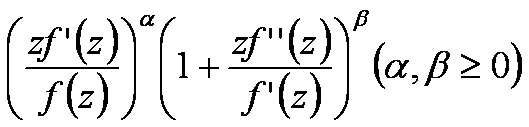lies in a region starlike with respect to 1 and symmetric with respect to the real axis. Also certain application of our main result for a class of functions defined by Hadamard product (convolution) is given. As a special case of our result we obtain Fekete-Szegö inequality for a class of functions defined through fractional derivatives. Also we obtain Fekete-Szegö inequality for the inverse functions.

1: Paper Source PDF document

Paper's Title:

Generalizations of two theorems on absolute summability methods

Author(s):

H.S. Özarslan and H.N. Öğdük

Department of Mathematics,
Erciyes University, 38039 Kayseri,
Turkey
seyhan@erciyes.edu.tr
nogduk@erciyes.edu.tr
URL: http://fef.erciyes.edu.tr/math/hikmet.htm

Abstract:

In this paper two theorems on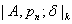summability methods, which generalize two theorems of Bor on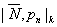summability methods, have been proved.

1: Paper Source PDF document

Paper's Title:

Oscillations of First Order Linear Delay Difference Equations

Author(s):

G. E. Chatzarakis and I. P. Stavroulakis

Department of Mathematics, University of Ioannina,
451 10, Greece
ipstav@cc.uoi.gr

Abstract:

Consider the first order linear delay difference equation of the form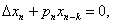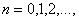where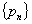is a sequence of nonnegative real numbers, k is a positive integer and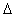denotes the forward difference operator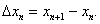New oscillation criteria are established when the well-known oscillation conditions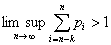and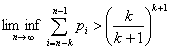are not satisfied. The results obtained essentially improve known results in the literature.

1: Paper Source PDF document

Paper's Title:

Analysis of the Flow Field in Stenosed Bifurcated Arteries Through a Mathematical Model

Author(s):

S. Chakravarty and S. Sen

Department of Mathematics, Visva-Bharati University,
Santiniketan 731235,
India
santabrata2004@yahoo.co.in

Abstract:

The present study is dealt with an appropriate mathematical model of the arotic bifurcation in the presence of constrictions using which the physiological flow field is analized. The geometry of the bifurcated arterial segment having constrictions in both the parent and its daughter arterial lumen frequently occurring in the diseased arteries causing malfunction of the cardiovascular system , is formed mathematically with the introduction of appropriate curvatures at the lateral junctions and the flow divider. The flowing blood contained in the stenosed bifurcated artery is treated to be Newtonian and the flow is considered to be two dimensional. The motion of the arterial wall and its effect on local fluid mechanics is not ruled out from the present pursuit. The flow analysis applies the time-dependent, two-dimensional incompressible nonlinear Navier-Stokes equations for Newtonian fluid. The flow field can be obtained primarily following the radial coordinate transformation and using the appropriate boundary conditions and finally adopting a suitable finite difference scheme numerically. The influences of the arterial wall distensibility and the presence of stenosis on the flow field, the flow rate and the wall shear stresses are quantified in order to indicate the susceptibility to atherosclerotic lesions and thereby to validate the applicability of the present theoretical model.

1: Paper Source PDF document

Paper's Title:

Note on the Rank of Birkhoff Interpolation

Author(s):

J. Rubió-Massegú

Applied Mathematics III, Universitat Politčcnica de Catalunya,
Colom 1, 08222, Terrassa,
Spain
josep.rubio@upc.edu

Abstract:

The relationship between a variant of the rank of a univariate Birkhoff interpolation problem, called normal rank, and other numbers of interest associated to the interpolation problem is studied.

1: Paper Source PDF document

Paper's Title:

On the Generalized Inverse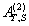over Integral Domains

Author(s):

Yaoming Yu and Guorong Wang

College of Education, Shanghai Normal University
Shanghai 200234
People's Republic of China.
yuyaoming@online.sh.cn
grwang@shnu.edu.cn

Abstract:

In this paper, we study further the generalized inverseof a matrix A over an integral domain. We give firstly some necessary and sufficient conditions for the existence of the generalized inverse, an explicit expression for the elements of the generalized inverseand an explicit expression for the generalized inverse, which reduces to the {1} inverse. Secondly, we verify that the group inverse, the Drazin inverse, the Moore-Penrose inverse and the weighted Moore-Penrose inverse are identical with the generalized inverse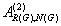for an appropriate matrix G, respectively, and then we unify the conditions for the existence and the expression for the elements of the weighted Moore-Penrose inverse, the Moore-Penrose inverse, the Drazin inverse and the group inverse over an integral domain. Thirdly, as a simple application, we give the relation between some rank equation and the existence of the generalized inverse, and a method to compute the generalized inverse. Finally, we give an example of evaluating the elements ofwithout calculating.

1: Paper Source PDF document

Paper's Title:

Construction of Lyapunov Functionals In Functional Differential Equations With Applications To Exponential Stability In Volterra Integro-differential Equations

Author(s):

Youssef N. Raffoul

Department of Mathematics, University of Dayton,
Dayton OH 45469-2316,
USA
youssef.raffoul@notes.udayton.edu

Abstract:

Non-negative definite Lyapunov functionals are employed to obtain sufficient conditions that guarantee the exponential asymptotic stability and uniform exponential asymptotic stability of the zero solution of nonlinear functional differential systems. The theory is applied to Volterra integro-differential equations in the form of proposition examples.

1: Paper Source PDF document

Paper's Title:

On Pseudo Almost Periodic Solutions to Some Neutral Functional-Differential Equations

Author(s):

Toka Diagana and Eduardo Hernández

Department of Mathematics, Howard University
2441 6th Street NW, Washington DC 20059,
USA.
tdiagana@howard.edu

Departamento de Matemática, I.C.M.C. Universidade de Săo Paulo,
Caixa Postal 668, 13560-970, Săo Carlos SP,
Brazil.
lalohm@icmc.sc.usp.br

Abstract:

This paper discusses the existence and uniqueness of pseudo almost periodic solutions to a class of partial neutral functional-differential equations. Under some suitable assumptions, existence and uniqueness results are obtained. An example is given to illustrate abstract results.

1: Paper Source PDF document

Paper's Title:

Normalized Truncated Levy models applied to the study of Financial Markets

Author(s):

M. C. Mariani, K. Martin, D. W. Dombrowski and D. Martinez

Department of Mathematical Sciences and Department of Finance,
New Mexico State University, P.O. Box 30001
Department 3MB Las Cruces, New Mexico 88003-8001
USA.
mmariani@nmsu.edu
kjmartin@nmsu.edu

Abstract:

This work is devoted to the study of the statistical properties of financial instruments from developed markets. We performed a new analysis of the behavior of companies corresponding to the DJIA index, and of the index itself, by using a normalized Truncated Levy walk model. We conclude that the Truncated Levy distribution describes perfectly the evolution of the companies and of the index near a crash.

1: Paper Source PDF document

Paper's Title:

On Sandwich Theorems for Certain Subclass of Analytic Functions Involving Dziok-Srivastava Operator

Author(s):

T. N. Shanmugam, M. P. Jeyarama and A. Singaravelu

Department of Mathematics
College of Engineering, Anna University
Chennai - 600 025,
India
drtns2001@yahoo.com

Department of Mathematics
Easwari Engineering College
Ramapuram, Chennai - 600089
jeyaraman-mp@yahoo.co.i

Department of Mathematics
Valliammai Engineering College
Chennai - 603203
asing-59@yahoo.com

Abstract:

The purpose of this present paper is to derive some subordination and superordination results for certain normalized analytic functions in the open unit disk, acted upon by Dziok-Srivastava operator. Relevant connections of the results, which are presented in this paper, with various known results are also considered.

1: Paper Source PDF document

Paper's Title:

On a Subclass of Uniformly Convex Functions Defined by the Dziok-Srivastava Operator

Author(s):

M. K. Aouf and G. Murugusundaramoorthy

Mathematics Department, Faculty of Science,
Mansoura University 35516,
Egypt.
mkaouf127@yahoo.com

School of Science and Humanities, VIT University
Vellore - 632014,
India.
gmsmoorthy@yahoo.com

Abstract:

Making use of the Dziok-Srivastava operator, we define a new subclass Tlm([α1];α,β) of uniformly convex function with negative coefficients. In this paper, we obtain coefficient estimates, distortion theorems, locate extreme points and obtain radii of close-to-convexity, starlikeness and convexity for functions belonging to the class Tlm([α1];α,β) . We consider integral operators associated with functions belonging to the class Hlm([α1];α,β) defined via the Dziok-Srivastava operator. We also obtain several results for the modified Hadamard products of functions belonging to the class Tlm([α1];α,β) and we obtain properties associated with generalized fractional calculus operators.

1: Paper Source PDF document

Paper's Title:

Some Inequalities for a Certain Class of Multivalent Functions Using Multiplier Transformation

Author(s):

Department Of Applied Mathematics
Sri Venkateswara College Of Engineering
Sriperumbudur, Chennai - 602105,
India.
suchithravenkat@yahoo.co.in

Department Of Mathematics,
Chennai - 600059,
India.

Department Of Applied Mathematics
Sri Venkateswara College Of Engineering
Sriperumbudur, Chennai - 602105,
India.
ganga@svce.ac.in

Department Of Mathematics,
Easwari Engineering College
Ramapuram, Chennai - 600089,
India.
ganga@svce.ac.in

Abstract:

The object of the present paper is to derive several inequalities associated with differential subordinations between analytic functions and a linear operator defined for a certain family of p-valent functions, which is introduced here by means of a family of extended multiplier transformations. Some special cases and consequences of the main results are also considered.

1: Paper Source PDF document

Paper's Title:

Contact With Adhesion between a Deformable Body and a Foundation

Author(s):

B. Teniou and M. Sofonea

Laboratoire de Mathematiques Appliquées et Modélisation,
Université Mentouri, Constantine 25000,
Algeria
tenioubou2@yahoo.fr

Laboratoire de Mathématiques et Physiques pour les Systémes,
Univesité de Perpignan,
France.
sofonea@univ-perp.fr

Abstract:

The aim of this work is study a dynamic contact problem between a deformable body and a foundation where the deformations are supposed to be small. The contact is with adhesion and normal compliance. The behavior of this body is modeled by a nonlinear elastic-visco-plastic law. The evolution of bonding field is described by a nonlinear differential equation. We derive a variational formulation of the contact problem and we prove the existence and uniqueness of its solution. The proof is based on the construction of three intermediate problems and then we construct a contraction mapping whose unique fixed point will be the weak solution of the mechanical problem.

1: Paper Source PDF document

Paper's Title:

Integer Sums of Powers of Trigonometric Functions (MOD p), for prime p

Author(s):

G. J. Tee

Department of Mathematics, University of Auckland, Auckland,
New Zealand
tee@math.auckland.ac.nz

Abstract:

Many multi--parameter families of congruences (mod p) are found for integer sums of  qth powers of the trigonometric functions over various sets of equidistant arguments, where p is any prime factor of q. Those congruences provide sensitive tests for the accuracy of software for evaluating trigonometric functions to high precision.

1: Paper Source PDF document

Paper's Title:

An Improved Mesh Independence Principle for Solving Equations and their Discretizations using Newton's Method

Author(s):

Ioannis K. Argyros

Cameron university,
Department of Mathematics Sciences,
Lawton, OK 73505,
USA
iargyros@cameron.edu

Abstract:

We improve the mesh independence principle  which states that when Newton's method is applied to an equation on a Banach space as well as to their finite--dimensional discretization there is a difference of at most one between the number of steps required by the two processes to converge to within a given error tolerance. Here using a combination of Lipschitz and center Lipschitz continuity assumptions instead of just Lipschitz conditions we show that the minimum number of steps required can be at least as small as in earlier works. Some numerical examples are provided whereas our results compare favorably with earlier ones.

1: Paper Source PDF document

Paper's Title:

Asymptotic Distribution of Products of Weighted Sums of Dependent Random Variables

Author(s):

Y. Miao and J. F. Li

College of Mathematics and Information Science,
Henan Normal University
Henan, China
yumiao728@yahoo.com.cn

College of Mathematics and Information Science,
Henan Normal University, 453007
Henan, China.
junfen_li@yahoo.com.cn

Abstract:

In this paper we establish the asymptotic distribution of products of weighted sums of dependent positive random variable, which extends the results of Rempała and Wesołowski (2002).

1: Paper Source PDF document

Paper's Title:

Approximation of Derivatives in a Singularly Perturbed Second Order Ordinary Differential Equation with Discontinuous Terms Arising in Chemical Reactor Theory

Author(s):

R. Mythili Priyadharshini and N. Ramanujam

Department of Mathematics, Bharathidasan University,
Tiruchirappalli - 620 024, Tamilnadu, India.
matram2k3@yahoo.com
URL: http://www.bdu.ac.in/depa/science/ramanujam.htm

Abstract:

In this paper, a singularly perturbed second order ordinary differential equation with a discontinuous convection coefficient arising in chemical reactor theory is considered. A robust-layer-resolving numerical method is suggested. An ε-uniform global error estimate for the numerical solution and also to the numerical derivative are established. Numerical results are provided to illustrate the theoretical results.

1: Paper Source PDF document

Paper's Title:

Real Interpolation Methods and Quasilogarithmic Operators

Author(s):

Ming Fan

School of Industrial Technology and Management,
Dalarna University, 781 88 Borlänge, Sweden

fmi@du.se
URL: http://users.du.se/~fmi

Abstract:

The purpose of this paper is to deal with nonlinear quasilogarithmic operators, which possesses the uniformly bounded commutator property on various interpolation spaces in the sense of Brudnyi-Krugljak associated with the quasi-power parameter spaces. The duality, and the domain and range spaces of these operators are under consideration. Some known inequalities for the Lebesgue integration spaces and the trace classes are carried over to the non-commutative symmetric spaces of measurable operators affiliated with a semi-finite von Neumann algebra.

1: Paper Source PDF document

Paper's Title:

Solution of One Conjecture on Inequalities with Power-Exponential Functions.

Author(s):

Seiichi Manyama

Osaka University

Japan

manchanr4@gmail.com

Abstract:

In this paper, we prove the open inequality aea+beb aeb+bea for all positive real numbers a and b.

1: Paper Source PDF document

Paper's Title:

An Lp Inequality for `Self-Reciprocal' Polynomials. II

Author(s):

M. A. Qazi

Department of Mathematics,
Tuskegee University,
Tuskegee, Alabama 36088
U.S.A.

Abstract:

The main result of this paper is a sharp integral mean inequality for the derivative of a `self-reciprocal' polynomial.

1: Paper Source PDF document

Paper's Title:

Subordination Results Associated with Hadamard Product

Author(s):

S. Sivasubramanian, C. Ramachandran and B. A. Frasin

Department of Mathematics,
University College of Engineering,
Anna University,
Saram-604 307,
India

sivasaisastha@rediffmail.com

Department of Mathematics,
University College of Engineering,
Anna University,
Villupuram,
India

crjsp2004@yahoo.com

Department of Mathematics,
Al al-Bayt University,
P.O. Box: 130095 Mafraq,
Jordan

Abstract:

In the present investigation, we consider an unified class of functions of complex order using Hadamard's convolution. We obtain a necessary and sufficient condition for functions to be in these classes.

1: Paper Source PDF document

Paper's Title:

On Generalization of Hardy-type Inequalities

Author(s):

K. Rauf, S. Ponnusamy and J. O. Omolehin

Department of Mathematics,
University of Ilorin, Ilorin,
Nigeria
krauf@unilorin.edu.ng

Department of Mathematics,
Chennai- 600 036,
India
samy@iitm.ac.in

Department of Mathematics,
University of Ilorin, Ilorin,
Nigeria
omolehin_joseph@yahoo.com

Abstract:

This paper is devoted to some new generalization of Hardy-type integral inequalities and the reversed forms. The study is to determine conditions on which the generalized inequalities hold using some known hypothesis. Improvement of some inequalities are also presented.

1: Paper Source PDF document

Paper's Title:

Certain Coefficient Estimates for Bi-univalent Sakaguchi Type Functions

Author(s):

B. Srutha Keerthi, S. Chinthamani

Department of Applied Mathematics,
Sri Venkateswara College of Engineering,
Sriperumbudur, Chennai - 602105,
India

Abstract:

Estimates on the initial coefficients are obtained for normalized analytic functions f in the open unit disk with f and its inverse g = f-1 satisfying the conditions that zf'(z) / f(z) and zg'(z) / g(z) are both subordinate to a starlike univalent function whose range is symmetric with respect to the real axis. Several related classes of functions are also considered, and connections to earlier known results are made.

1: Paper Source PDF document

Paper's Title:

On the Sendov Conjecture for a Root Close to the Unit Circle

Author(s):

Indraneel G. Kasmalkar

Department of Mathematics,
University of California,
Berkeley, CA 94720
United States of America

E-mail: indraneelk@berkeley.edu

Abstract:

On Sendov's conjecture, T. Chijiwa quantifies the idea stated by V. Vâjâitu and A. Zaharescu (and M. J. Miller independently), namely that if a polynomial with all roots inside the closed unit disk has a root sufficiently close to the unit circle then there is a critical point at a distance of at most one from that root. Chijiwa provides an estimate of exponential order for the required 'closeness' of the root to the unit circle so that such a critical point may exist. In this paper, we will improve this estimate to polynomial order by making major modifications and strengthening inequalities in Chijiwa's proof.

1: Paper Source PDF document

Paper's Title:

L∞- Error Estimate of Schwarz Algorithm for Elliptic Quasi-Variational Inequalities Related to Impulse Control Problem

Author(s):

Lab. LANOS, Department of Mathematics,
P.O.Box 12, Annaba 23000,
Algeria.

Lab. LAIG, Department of Mathematics,
University May 8th 1945,
P.O.Box 401, Guelma 24000,
Algeria.

allmehri@yahoo.fr

Abstract:

In this work, we study Schwarz method for a class of elliptic quasi-variational inequalities. The principal result of this investigation is to prove the error estimate in ∞-norm for two domains with overlapping nonmatching grids, using the geometrical convergence, and the uniform convergence of Cortey Dumont.

1: Paper Source PDF document

Paper's Title:

Weak solutions of non coercive stochastic Navier-Stokes equations in R2

Author(s):

Wilhelm Stannat and Satoshi Yokoyama

Technische Universität Berlin,
Strasse des 17. Juni 136, 10623 Berlin,
Germany.

The University of Tokyo,
Komaba, Tokyo 153-8914,
Japan.

E-mail: stannat@math.tu-berlin.de

E-mail: satoshi2@ms.u-tokyo.ac.jp

Abstract:

We prove existence of weak solutions of stochastic Navier-Stokes equations in R2 which do not satisfy the coercivity condition. The equations are formally derived from the critical point of some variational problem defined on the space of volume preserving diffeomorphisms in R2. Since the domain of our equation is unbounded, it is more difficult to get tightness of approximating sequences of solutions in comparison with the case of a bounded domain. Our approach is based on uniform a priori estimates on the enstrophy of weak solutions of the stochastic 2D-Navier-Stokes equations with periodic boundary conditions, where the periodicity is growing to infinity combined with a suitable spatial cutoff-technique.

1: Paper Source PDF document

Paper's Title:

Sufficient Conditions for Certain Types of Functions to be Parabolic Starlike

Author(s):

Department of Mathematics,
Easwari Engineering College,
Ramapuram, Chennai - 89,
India.

Research Scholar,
Anna University,
Chennai

E-mail: ganga.megalai@gmail.com

E-mail: chinvicky@rediffmail.com

Abstract:

In this paper sufficient conditions are determined for functions of the form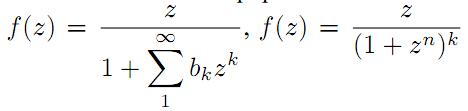and certain other types of functions to be parabolic starlike.

1: Paper Source PDF document

Paper's Title:

Some New Generalizations of Jensen's Inequality with Related Results and Applications

Author(s):

Steven G. From

Department of Mathematics

E-mail: sfrom@unomaha.edu

Abstract:

In this paper, some new generalizations of Jensen's inequality are presented. In particular, upper and lower bounds for the Jensen gap are given and compared analytically and numerically to previously published bounds for both the discrete and continuous Jensen's inequality cases. The new bounds compare favorably to previously proposed bounds. A new method based on a series of locally linear interpolations is given and is the basis for most of the bounds given in this paper. The wide applicability of this method will be demonstrated. As by-products of this method, we shall obtain some new Hermite-Hadamard inequalities for functions which are 3-convex or 3-concave. The new method works to obtain bounds for the Jensen gap for non-convex functions as well, provided one or two derivatives of the nonlinear function are continuous. The mean residual life function of applied probability and reliability theory plays a prominent role in construction of bounds for the Jensen gap. We also present an exact integral representation for the Jensen gap in the continuous case. We briefly discuss some inequalities for other types of convexity, such as convexity in the geometric mean, and briefly discuss applications to reliability theory.

1: Paper Source PDF document

Paper's Title:

Bartle Integration in Lie Algebras

Author(s):

Andreas Boukas and Philip Feinsilver

Centro Vito Volterra,
Universita di Roma Tor Vergata,
via Columbia 2, 00133 Roma,
Italy.

Department of Mathematics,
Southern Illinois University,
Carbondale, Illinois 62901,
USA.

E-mail: andreasboukas@yahoo.com
E-mail:  pfeinsil@math.siu.edu

Abstract:

Using Bartle's bilinear vector integral we define stochastic integrals of bounded operator valued functions with respect to Stieltjes measures associated with the generators of the Heisenberg and Finite Difference Lie algebras. Our definition also covers the Square of White Noise and sl/2 Lie algebras.

1: Paper Source PDF document

Paper's Title:

Hyponormal and K-Quasi-Hyponormal Operators On Semi-Hilbertian Spaces

Author(s):

Ould Ahmed Mahmoud Sid Ahmed and Abdelkader Benali

Mathematics Department,
College of Science,
Aljouf University,
Aljouf 2014,
Saudi Arabia.
E-mail: sididahmed@ju.edu.sa

Mathematics Department, Faculty of Science,
Hassiba Benbouali, University of Chlef,
B.P. 151 Hay Essalem, Chlef 02000,
Algeria.
E-mail: benali4848@gmail.com

Abstract:

Let H be a Hilbert space and let A be a positive bounded operator on H. The semi-inner product < u|v>A:=<Au|v>, u,v H induces a semi-norm || .||A on H. This makes H into a semi-Hilbertian space. In this paper we introduce the notions of hyponormalities and k-quasi-hyponormalities for operators on semi Hilbertian space (H,||.||A), based on the works that studied normal, isometry, unitary and partial isometries operators in these spaces. Also, we generalize some results which are already known for hyponormal and quasi-hyponormal operators. An operator T BA (H) is said to be (A, k)-quasi-hyponormal if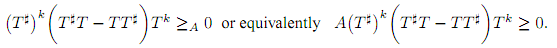1: Paper Source PDF document

Paper's Title:

The boundedness of Bessel-Riesz operators on generalized Morrey spaces

Author(s):

Mochammad Idris, Hendra Gunawan and Eridani

Department of Mathematics,
Bandung Institute of Technology,
Bandung 40132,
Indonesia.
E-mail: mochidris@students.itb.ac.id

Department of Mathematics,
Bandung Institute of Technology,
Bandung 40132,
Indonesia.
E-mail: hgunawan@math.itb.ac.id
URL: http://personal.fmipa.itb.ac.id/hgunawan/

Department of Mathematics,
Airlangga University,
Surabaya 60115,
Indonesia.

Abstract:

In this paper, we prove the boundedness of Bessel-Riesz operators on generalized Morrey spaces. The proof uses the usual dyadic decomposition, a Hedberg-type inequality for the operators, and the boundedness of Hardy-Littlewood maximal operator. Our results reveal that the norm of the operators is dominated by the norm of the kernels.

1: Paper Source PDF document

Paper's Title:

Ostrowski Type Inequalities for Lebesgue Integral: a Survey of Recent Results

Author(s):

Sever S. Dragomir1,2

1Mathematics, School of Engineering & Science
Victoria University, PO Box 14428
Melbourne City, MC 8001,
Australia
E-mail: sever.dragomir@vu.edu.au

2DST-NRF Centre of Excellence in the Mathematical and Statistical Sciences,
School of Computer Science & Applied Mathematics,
University of the Witwatersrand,
Private Bag 3, Johannesburg 2050,
South Africa
URL: http://rgmia.org/dragomir

Abstract:

The main aim of this survey is to present recent results concerning Ostrowski type inequalities for the Lebesgue integral of various classes of complex and real-valued functions. The survey is intended for use by both researchers in various fields of Classical and Modern Analysis and Mathematical Inequalities and their Applications, domains which have grown exponentially in the last decade, as well as by postgraduate students and scientists applying inequalities in their specific areas.

1: Paper Source PDF document

Paper's Title:

On Singular Numbers of Hankel Matrices of Markov Functions

Author(s):

Vasily A. Prokhorov

Department of Mathematics and Statistics,
University of South Alabama,
Mobile, Alabama 36688-0002,
USA.
E-mail: prokhoro@southalabama.edu
URL: http://www.southalabama.edu/mathstat/people/prokhorov.shtml

Abstract:

Let E ⊂ (01,1) be a compact set and let μ be a positive Borel measure with support supp μ=E. Let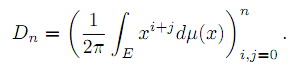In the case when E=[a,b]⊂ (-1,1) and μ satisfies the condition dμ/dx>0 a.e. on E, we investigate asymptotic behavior of singular numbers σkn,n of the Hankel matrix Dn, where kn/n→θ∈[0,1] as n→∞. Moreover, we obtain asymptotics of the Kolmogorov, Gelfand and linear k-widths, k=kn, of the unit ball An,2 of Pn∩L2(Γ) in the space L2(μ,E), where Γ={z:|z|=1} and Pn is the class of all polynomials of the degree at most n.

1: Paper Source PDF document

Paper's Title:

Polyanalytic Functions on Subsets of Z[i]

Author(s):

Abtin Daghighi

SE-581 83,
Sweden.

E-mail: abtindaghighi@gmail.com

Abstract:

For positive integers q we consider the kernel of the powers Lq where L is one of three kinds of discrete analogues of the Cauchy-Riemann operator. The first two kinds are well-studied, but the third kind less so. We give motivations for further study of the third kind especially since its symmetry makes it more appealing for the cases q 2.

From an algebraic perspective it makes sense that the chosen multiplication on the kernels is compatible with the choice of pseudo-powers. We propose such multiplications together with associated pseudo-powers. We develop a proof-tool in terms of certain sets of uniqueness.

1: Paper Source PDF document

Paper's Title:

A Comparison Between Two Different Stochastic Epidemic Models with Respect to the Entropy

Author(s):

Department of Mathematics,
University of Sussex,
Brighton BN1 9QH,
UK.
E-mail: f.fatehi@sussex.ac.uk
URL: http://www.sussex.ac.uk/profiles/361251

Department of Pure Mathematics, Faculty of Mathematics and Computer,
Shahid Bahonar University of Kerman,
Kerman 76169-14111,
Iran.

Abstract:

In this paper at first a brief history of mathematical models is presented with the aim to clarify the reliability of stochastic models over deterministic models. Next, the necessary background about random variables and stochastic processes, especially Markov chains and the entropy are introduced. After that, entropy of SIR stochastic models is computed and it is proven that an epidemic will disappear after a long time. Entropy of a stochastic mathematical model determines the average uncertainty about the outcome of that random experiment. At the end, we introduce a chain binomial epidemic model and compute its entropy, which is then compared with the DTMC SIR epidemic model to show which one is nearer to reality.

1: Paper Source PDF document

Paper's Title:

A Generalization of Ostrowski's Inequality for Functions of Bounded Variation via a Parameter

Author(s):

Seth Kermausuor

Department of Mathematics and Computer Science,
Alabama State University,
Montgomery, AL 36101,
USA.
E-mail: skermausour@alasu.edu

Abstract:

In this paper, we provide a generalization of the Ostrowski's inequality for functions of bounded variation for k points via a parameter λ∈[0,1]. As a by product, we consider some particular cases to obtained some interesting inequalities in these directions. Our results generalizes some of the results by Dragomir in [S. S. DRAGOMIR, The Ostrowski inequality for mappings of bounded variation, Bull. Austral. Math. Soc., 60 (1999), pp. 495--508.]

1: Paper Source PDF document

Paper's Title:

On a subset of Bazilevic functions

Author(s):

Marjono and D. K. Thomas

Department of Mathematics,
Faculty of Mathematics and Natural Sciences,
Brawajaya University,
Malang, Jawa Timur 65145,
Indonesia.
E-mail: marjono@ub.ac.id

Department of Mathematics,
Swansea University, Singleton Park,
Swansea, SA2 8PP,
United Kingdom.
E-mail: d.k.thomas@swansea.ac.uk

Abstract:

Let S denote the class of analytic and univalent functions in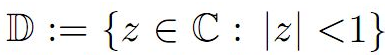of the form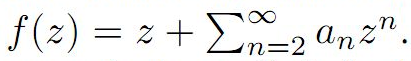For α≥0, the subclass B1α of S of Bazilevic functions has been extensively studied. In this paper we determine various properties of a subclass of B1α, for α≥0 which extends early results of a class of starlike functions studied by Ram Singh.

1: Paper Source PDF document

Paper's Title:

Countable Ordinal Spaces and Compact Countable Subsets of a Metric Space

Author(s):

B. Alvarez-Samaniego, A. Merino

Quito,
E-mail: borys_yamil@yahoo.com, balvarez@uce.edu.ec

Escuela de Ciencias Fisicas y Matematica
Facultad de Ciencias Exactas y Naturales
E-mail: aemerinot@puce.edu.ec

Abstract:

We show in detail that every compact countable subset of a metric space is homeomorphic to a countable ordinal number, which extends a result given by Mazurkiewicz and Sierpinski for finite-dimensional Euclidean spaces. In order to achieve this goal, we use Transfinite Induction to construct a specific homeomorphism. In addition, we prove that for all metric space, the cardinality of the set of all the equivalence classes, up to homeomorphisms, of compact countable subsets of this metric space is less than or equal to aleph-one. We also show that for all cardinal number smaller than or equal to aleph-one, there exists a metric space with cardinality equals the aforementioned cardinal number.

1: Paper Source PDF document

Paper's Title:

Applications of Von Neumann Algebras to Rigidity Problems of (2-Step) Riemannian (Nil-)Manifolds

Author(s):

DFouman Faculty of Engineering,
College of Engineering, University of Tehran,
Iran.

Department of Mathematical Sciences,
Sharif University of Technology,
Iran
E-mail: fanai@sharif.edu

Abstract:

In this paper, basic notions of von Neumann algebra and its direct analogues in the realm of groupoids and measure spaces have been considered. By recovering the action of a locally compact Lie group from a crossed product of a von Neumann algebra, other proof of one of a geometric propositions of O'Neil and an extension of it has been proposed. Also, using the advanced exploration of nilmanifolds in measure spaces and their corresponding automorphisms (Lie algebraic derivations) a different proof of an analytic theorem of Gordon and Mao has been attained. These two propositions are of the most important ones for rigidity problems of Riemannian manifolds especially 2-step nilmanifolds.

1: Paper Source PDF document

Paper's Title:

Simplicial (co)-homology of Band Semigroup

Author(s):

Yasser Farhat

Abu Dhabi Polytechnic,
P.O. Box 111499, Abu Dhabi,
UAE.

Abstract:

We consider the Banach algebra l1(S), with convolution, where S is a band semigroup. We prove directly, without using the cyclic cohomology, that the simplicial cohomology groups Hn(l1(S), l1(S)*) vanish for all n1. This proceeds in three steps. In each step, we introduce a bounded linear map. By iteration in each step, we achieve our goal.

1: Paper Source PDF document

Paper's Title:

The Higher Coefficients for Bazilevic Functions B1(α)

Author(s):

Department of Mathematics,
Faculty of Mathematics and Natural Sciences,,
Brawijaya University, Malang Jawa Timur 65145
Indonesia.
E-mail: marjono@ub.ac.id

Abstract:

Let f be analytic in D{z: |z|< 1} with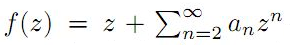, and normalized by the conditions f(0)=f'(0)-1=0. We give sharp estimates for the seventh and eighth coefficients for the class of Bazilevic functions with logarithmic growth, B1α, defined by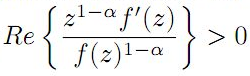for α≥0.

1: Paper Source PDF document

Paper's Title:

Numerical Approximation by the Method of Lines with Finite-volume Approach of a Solute Transport Equation in Periodic Heterogeneous Porous Medium

Author(s):

D. J. Bambi Pemba and B. Ondami

Université Marien Ngouabi,
Factuté des Sciences et Techniques,
BP 69, Brazzaville,
Congo.
E-mail: bondami@gmail.com

Abstract:

In this paper we are interested in the numerical approximation of a two-dimensional solute transport equation in heterogeneous porous media having periodic structures. It is a class of problems which has been the subject of various works in the literature, where different methods are proposed for the determination of the so-called homogenized problem. We are interested in this paper, in the direct resolution of the problem, and we use the method of lines with a finite volume approach to discretize this equation. This discretization leads to an ordinary differential equation (ODE) that we discretize by the Euler implicit scheme. Numerical experiments comparing the obtained solution and the homogenized problem solution are presented. They show that the precision and robustness of this method depend on the ratio between, the mesh size and the parameter involved in the periodic homogenization.

1: Paper Source PDF document

Paper's Title:

Reduced Generalized Combination Synchronization Between Two n-Dimensional Integer-Order Hyperchaotic Systems and One m-Dimensional Fractional-Order Chaotic System

Author(s):

Smail Kaouache, Mohammed Salah Abdelouahab and Rabah Bououden

Laboratory of Mathematics and their interactions,
Abdelhafid Boussouf University Center, Mila.
Algeria
E-mail: smailkaouache@gmail.com, medsalah3@yahoo.fr, rabouden@yahoo.fr

Abstract:

This paper is devoted to investigate the problem of reduced generalized combination synchronization (RGCS) between two n-dimensional integer-order hyperchaotic drive systems and one m-dimensional fractional-order chaotic response system. According to the stability theorem of fractional-order linear system, an active mode controller is proposed to accomplish this end. Moreover, the proposed synchronization scheme is applied to synchronize three different chaotic systems, which are the Danca hyperchaotic system, the modified hyperchaotic Rossler system, and the fractional-order Rabinovich-Fabrikant chaotic system. Finally, numerical results are presented to fit our theoretical analysis.

1: Paper Source PDF document

Paper's Title:

A Note on Schur's Lemma in Banach Function Spaces

Author(s):

R. E. Castillo, H. Rafeiro and E. M. Rojas

Departamento de Matematicas, Bogota,
Colombia.
E-mail: recastillo@unal.edu.co

United Arab Emirates University,
Department of Mathematical Sciences, Al Ain,
United Arab Emirates.
E-mail: rafeiro@uaeu.ac.ae

Departamento de Matematicas, Bogota,
Colombia.
E-mail: emrojass@unal.edu.co

Abstract:

In this small note, in a self contained presentation, we show the validity of Schur's type lemma in the framework of Banach function spaces.

1: Paper Source PDF document

Paper's Title:

Lie Group Theoretic Approach of One-Dimensional Black-Scholes Equation

Author(s):

P. L. Zondi and M. B. Matadi

Department of Mathematical sciences,
Faculty of Sciences & Agriculture, University of Zululand,
P Bag X1001, Kwa-Dlangezwa 3886,
South Africa.
zondip@unizulu.ac.za

Abstract:

This study discusses the Lie Symmetry Analysis of Black-Scholes equation via a modified local one-parameter transformations. It can be argued that the transformation of the Black-Scholes equation is firstly obtained by means of riskless rate. Thereafter, the corresponding determining equations to the reduced equation are found. Furthermore, new symmetries of the Black-Scholes equation are constructed and lead to invariant solutions.

1: Paper Source PDF document

Paper's Title:

Hankel Functional Connected to Lemniscate of Bernoulli

Author(s):

K. Ramanuja Rao, Rajnesh Lal and Kaushal Singh

Fiji National University,
Department of Mathematics & Statistics,
P.O. Box 5529, Lautoka,
Fiji.
E-mail: ramanuja.kotti@fnu.ac.fj
rajnesh.lal@fnu.ac.fj
kaushal.singh@fnu.ac.fj

Abstract:

The aim of present paper is to derive a higher bound (HB) of 3rd order Hankel determinant for a collection of holomorphic mappings connected with exactly to the right side of the lemniscate of Bernoulli, whose polar coordinates form is r2 = 2cos2(2θ). The method carried in this paper is more refined than the method adopted by the authors (see ), who worked on this problem earlier.

1: Paper Source PDF document

Paper's Title:

Locally Bicomplex Convex Module and Their Applications

Author(s):

Department of Mathematics,
University of Jammu,
Jammu And Kashmir,
India.
E-mail: stanzinkunga19@gmail.com

Department of Mathematics,
University of Jammu,
Jammu And Kashmir,
India.

Abstract:

Let X be a locally BC convex module and L(X) be the family of all continuous bicomplex linear operators on X. In this paper, we study some concepts of D-valued seminorms on locally BC convex module. Further, we study the bicomplex version of Co and (Co,1) semigroup. The work of this paper is inspired by the work in  and .

1: Paper Source PDF document

Paper's Title:

An Integration Technique for Evaluating Quadratic Harmonic Sums

Author(s):

J. M. Campbell and K.-W. Chen

Department of Mathematics and Statistics,
York University, 4700 Keele St, Toronto,
ON M3J 1P3,
E-mail: jmaxwellcampbell@gmail.com

Department of Mathematics, University of Taipei,
Taipei 10048, Taiwan.
E-mail: kwchen@uTaipei.edu.tw
URL: https://math.utaipei.edu.tw/p/412-1082-22.php

Abstract:

The modified Abel lemma on summation by parts has been applied in many ways recently to determine closed-form evaluations for infinite series involving generalized harmonic numbers with an upper parameter of two. We build upon such results using an integration technique that we apply to ``convert'' a given evaluation for such a series into an evaluation for a corresponding series involving squared harmonic numbers.

1: Paper Source PDF document

Paper's Title:

A Caratheodory's Approximate Solutions of Stochastic Differential Equations Under the Hölder Condition

Author(s):

Bo-Kyeong Kim and Young-Ho Kim

Department of Mathematics,
Changwon National University,
Changwon, Gyeongsangnam-do 51140,
Korea.
E-mail: claire9576@naver.com
yhkim@changwon.ac.kr

Abstract:

In this paper, based on the theorem of the uniqueness of the solution of the stochastic differential equation, the convergence possibility of the Caratheodory's approximate solution was studied by approximating the unique solution. To obtain this convergence theorem, we used a Hölder condition and a weakened linear growth condition. Furthermore, The auxiliary theorems for the existence and continuity of the Caratheodory's approximate solution were investigated as a prerequisite.

1: Paper Source PDF document

Paper's Title:

Introducing the Picard-S3 Iteration for Fixed Points

Author(s):

Pravin Singh, Virath Singh and Shivani Singh

University of KwaZulu-Natal,
School of Mathematics Statistics and Computer Sciences,
Private Bag X54001,
Durban, 4000
South Africa.

Unisa,
Department of Decision Sciences,
PO Box 392,
Pretoria, 0003
South Africa.
E-mail: singhprook@gmail.com
singhv@ukzn.ac.za
shivstarsingh@gmail.com

Abstract:

In this paper we introduce a three step iteration method and show that it can be used to approximate the fixed point of a weak contraction mapping. Furthermore, we prove that this scheme is equivalent to the Mann iterative scheme. A comparison is made with other three step iterative methods by examining the speed of convergence. Results are presented in tables to support our conclusion.

1: Paper Source PDF document

Paper's Title:

Fekete Szegö problem on the Class of Bazilevič functions B1(α) related to the Lemniscate Bernoulli

Author(s):

N. M. Asih, Marjono, Sa'adatul Fitri, Ratno Bagus Edy Wibowo

Department of Mathematics,
University of Brawijaya,
Malang 65145,
Indonesia.
Department of Mathematics,
University of Udayana,
Bali,
Indonesia.

Department of Mathematics,
University of Brawijaya,
Malang 65145,
Indonesia.
E-mail: marjono@ub.ac.id

Department of Mathematics,
University of Brawijaya,
Malang 65145,
Indonesia.

Department of Mathematics,
University of Brawijaya,
Malang 65145,
Indonesia.
E-mail: rbagus@ub.ac.id

Abstract:

We provide a sharp boundaries inequalities for Fekete Szegö problem |a3-μ a22|, the coefficients of logarithmic function log~ f(z)/z, and the coefficients of the inverse function f(f'(w)) on the Bazilevič functions B1(α) related to the Lemniscate Bernoulli on the unit disk D={z: |z| < 1}. We obtained the result by using some properties of function with positive real part relates to coefficients problems.

1: Paper Source PDF document

Paper's Title:

Bicomplex Univalent Functions

Author(s):

Mohd Arif, Amjad Ali, Rajat Singh* and Romesh Kumar

Department of Mathematics,
University of Jammu,
Jammu And Kashmir,
India.
E-mail: azizymaths@gmail.com

Department of Mathematics,
University of Jammu,
Jammu And Kashmir,
India.

Department of Mathematics,
University of Jammu,
Jammu And Kashmir,
India.
E-mail: *rajat.singh.rs634@gmail.com

Department of Mathematics,
University of Jammu,
Jammu And Kashmir,
India.
E-mail: romeshmath@gmail.com

Abstract:

In this paper we introduce bicomplex univalent functions and also discuss the properties of a specific class of univalent functions.

Search and serve lasted 1 second(s).